# 什么是数据结构？

• 数据结构是计算机存储、组织数据的方式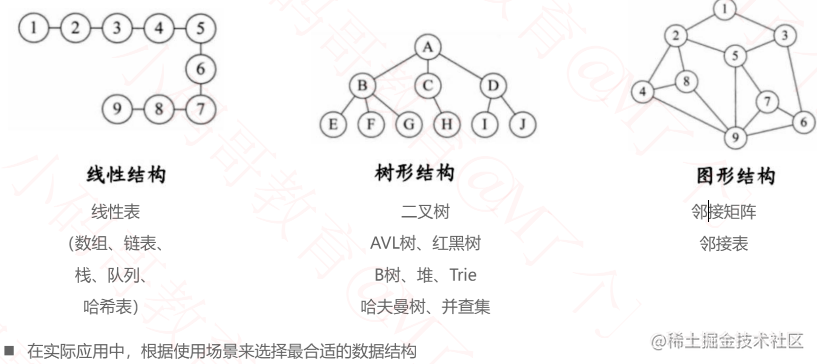# 一、线性结构, 线性表

• 线性表是具有n个相同类型元素的有限序列（n >= 0）
• 常见的线性表有: 数组、链表、栈、队列、哈希表(散列表)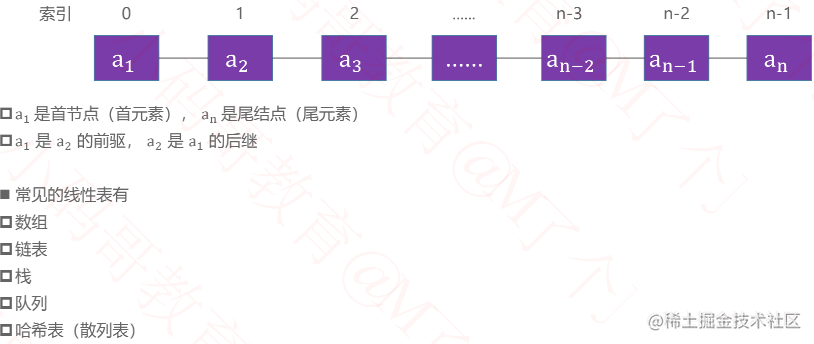## 基本概念

### 时间复杂度

• 大O表示法中, 时间复杂度的公式是: T(n) = O(f(n)), 其中f(n)表示每个代码执行次数之和, 而O表示正比例关系, 这个公式的全称是:算法的渐进时间复杂度.
• 常见的时间复杂度量级从上至下越来越大:
• 常数阶O(1)
• 对数阶O(logN)
• 线性对数阶O(nlogN)
• 平方阶O(n²)
• 立方阶O(n³)
• K次方阶O(n^k)
• 指数阶O(2^n)

### 空间复杂度

• 空间复杂度是对一个算法在运行过程中临时占用存储空间大小的一个量度, 同样反映的是一个趋势, 我们用S(n)来定义.
• 空间复杂度比较常用的有: O(1)、O(n)、O(n²).
• 如果算法执行所需要的临时空间不随着某个变量n的大小而变化, 即此算法空间复杂度为一个常量, 可表示为O(1) ```// 代码中的i、j、m所分配的空间都不随着处理数据量变化, 因此它的空间复杂度S(n) = O(1) int j = 1; int j = 2; ++i; j++; int m = i + j;```
• 空间复杂度O(n) ```int[] m = new int[n]; for (i = 1; i <= n; ++i) { j = i; j++ }```
• 这段代码中, 第一行new了一个数组出来, 这个数据占用的大小为n, 这段代码的2-6航, 虽然有循环, 但没有再分配新的空间, 因此, 这段代码的空间复杂度主要看第一行即可, 即S(n) = O(n);

## 1. 数组（Array）

• 数组是一种顺序存储的线性表， 所有元素的内存地址是连续的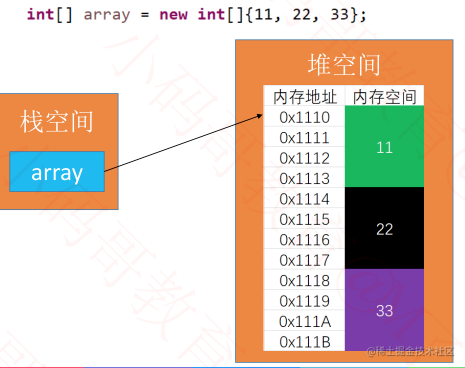• 很多编程语言中， 数组都有个缺点，无法动态修改容量
• 实际开发中， 我们更希望数组的容量是可以动态改变的

### 动态数组的接口设计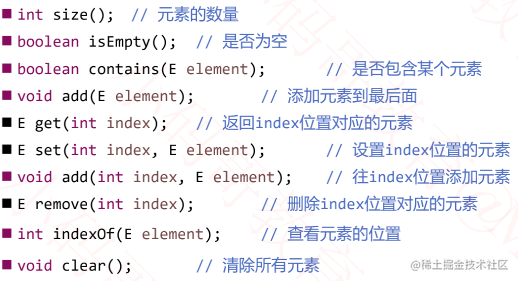#### 动态数组的设计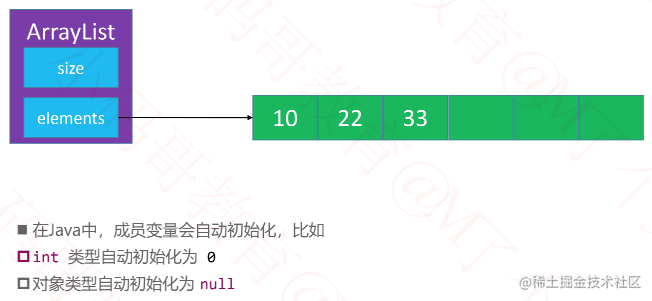- 添加元素 add(E element)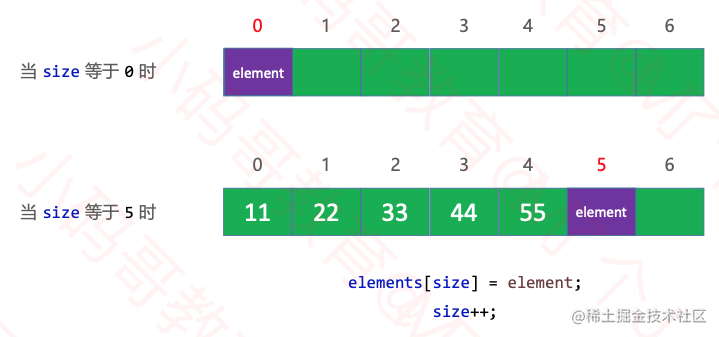• 打印数组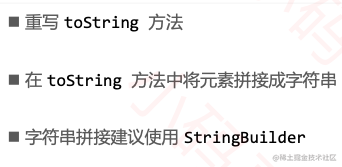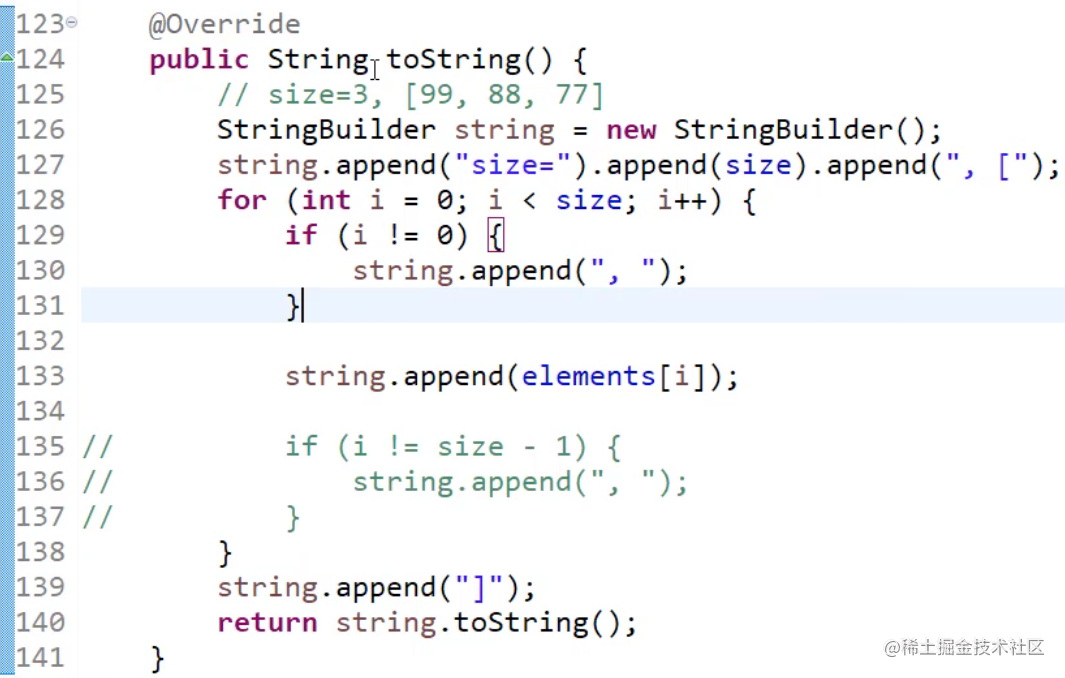• 删除元素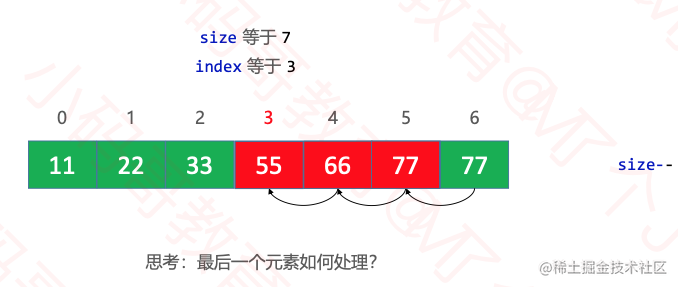- `new`申请的是连续的内存空间，不能中间挖掉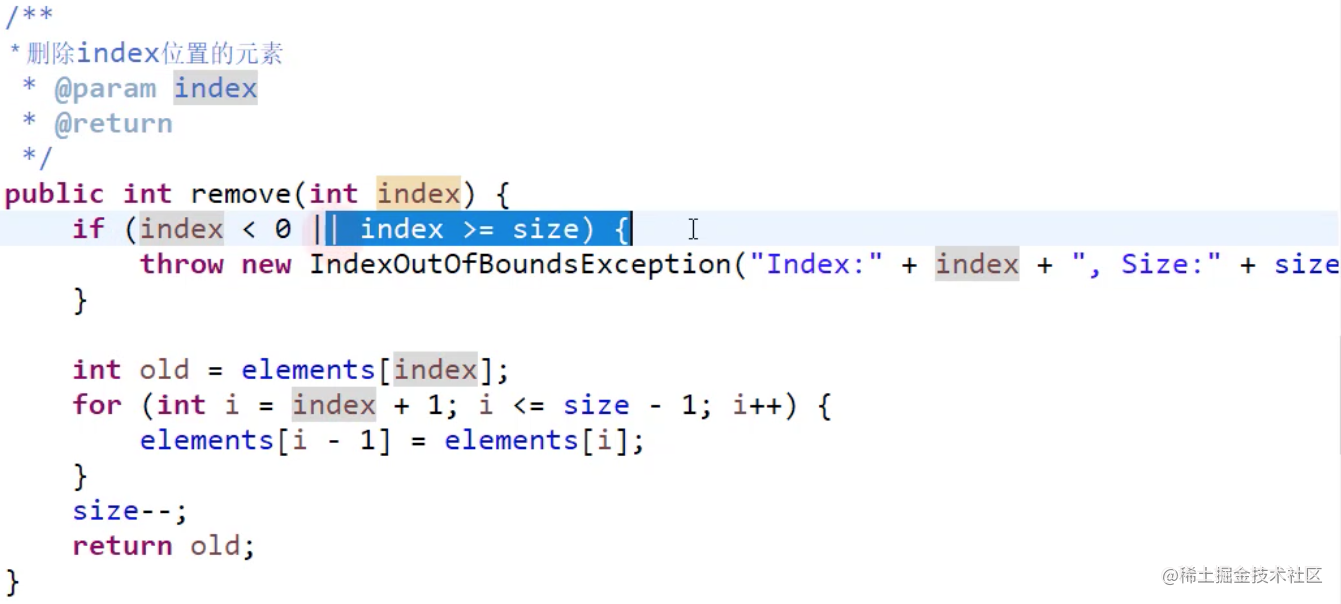• 插入添加元素 `add(int index, E element)`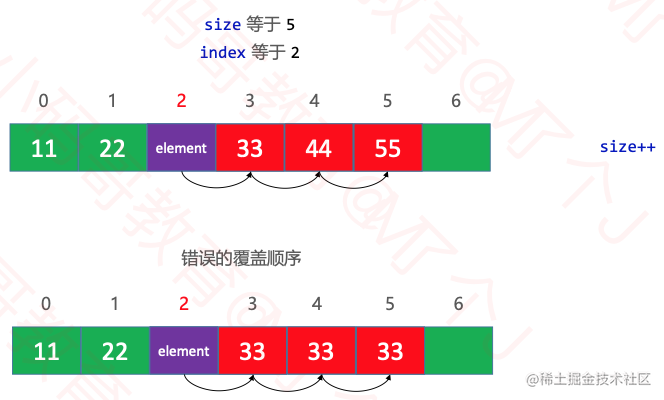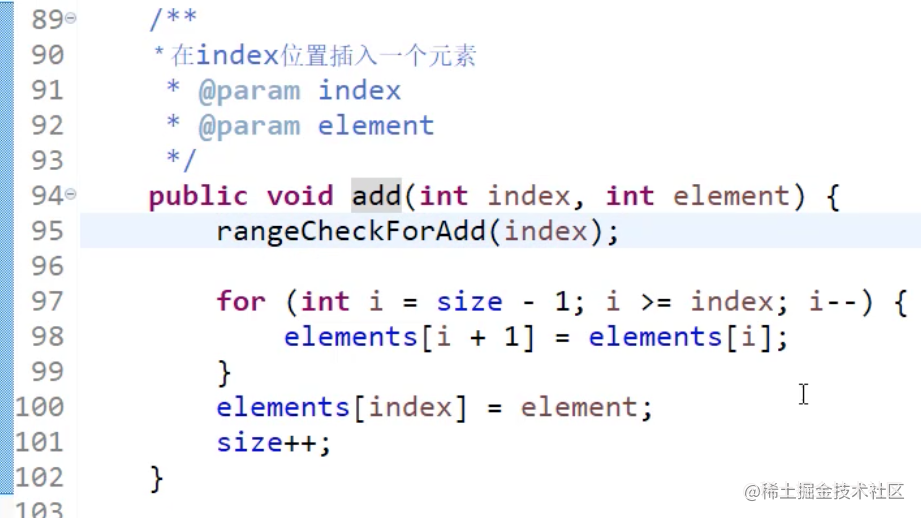- 把合规判断抽取出来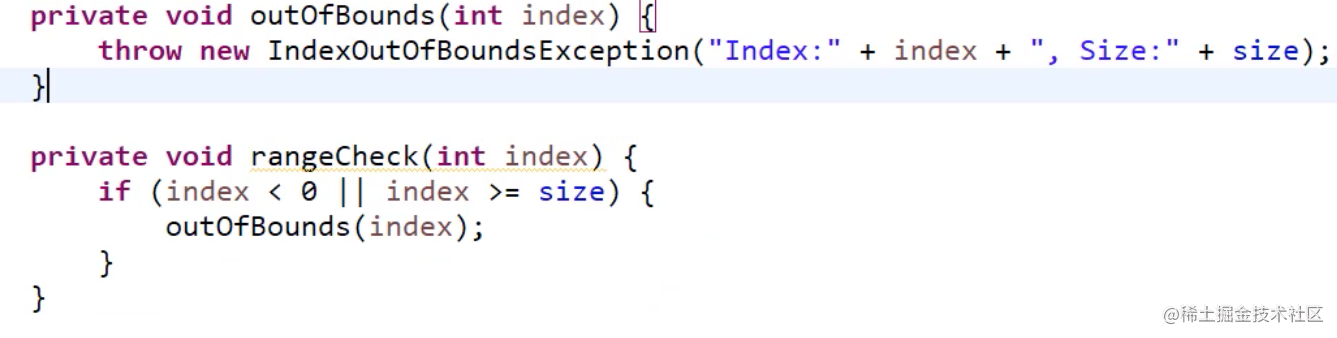• 动态数组有个明显的缺点
• 可能会造成内存空间的大量浪费
• 链表到多少内存就申请多少内存
• `链表`是一种`链式存储`线性表, 所有元素的内存地址不一定是连续的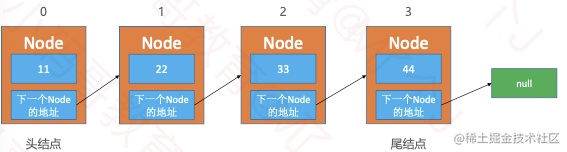### 反转链表

• 输入: 1->2->3->4->5->NULL
• 输出: NULL->5->4->3->2->1

#### 递归法翻转链表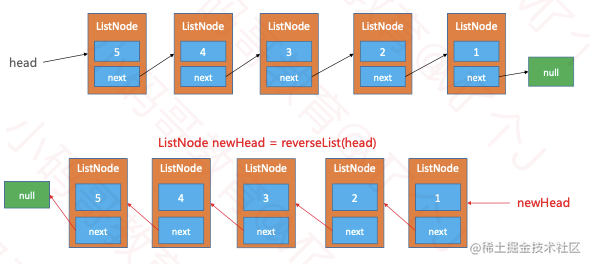• `ListNode newHead = reverseList(head.next)`做的事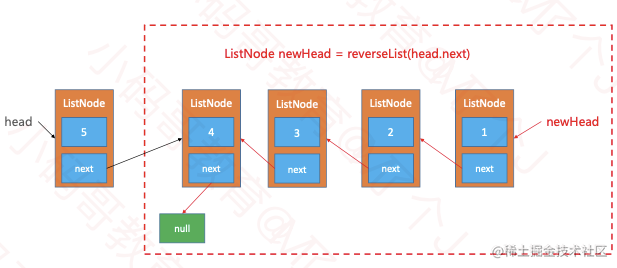``` // 递归的方式反转链表 Java: public ListNode reverseList(ListNode head) { // 1. 传入的参数合法性 || 递归的终止条件 if (head == null || head.next == null) return head;

}

Swift: /* * Definition for singly-linked list. * public class ListNode { * public var val: Int * public var next: ListNode? * public init() { self.val = 0; self.next = nil; } * public init( val: Int) { self.val = val; self.next = nil; } * public init( val: Int, _ next: ListNode?) { self.val = val; self.next = next; } * } / class Solution { func reverseList(_ head: ListNode?) -> ListNode? { // 采用递归的方式翻转链表 // 1. 传入的参数合法性 || 递归的终止条件 if head == nil || head?.next == nil { return head }

``````    // 2. 递归, 一直递归到链表的最后一个结点, 该结点就是反转后的头结点
// 3. 每次函数在返回过程中, 将当前结点的后一个结点的指针指向当前结点, 并断开后一个结点指向后方的指针
// 4. 断开当前结点指向后一个结点的指针, 并指向nil, 从而实现链表尾部开始的局部反转

// 5. 返回反转后的链表, 当递归函数全部出栈后, 链表反转完成.
}
``````

} ``` 反转链表力扣链接

### 双向循环链表

#### 双向循环链表添加 - add(int index, E element)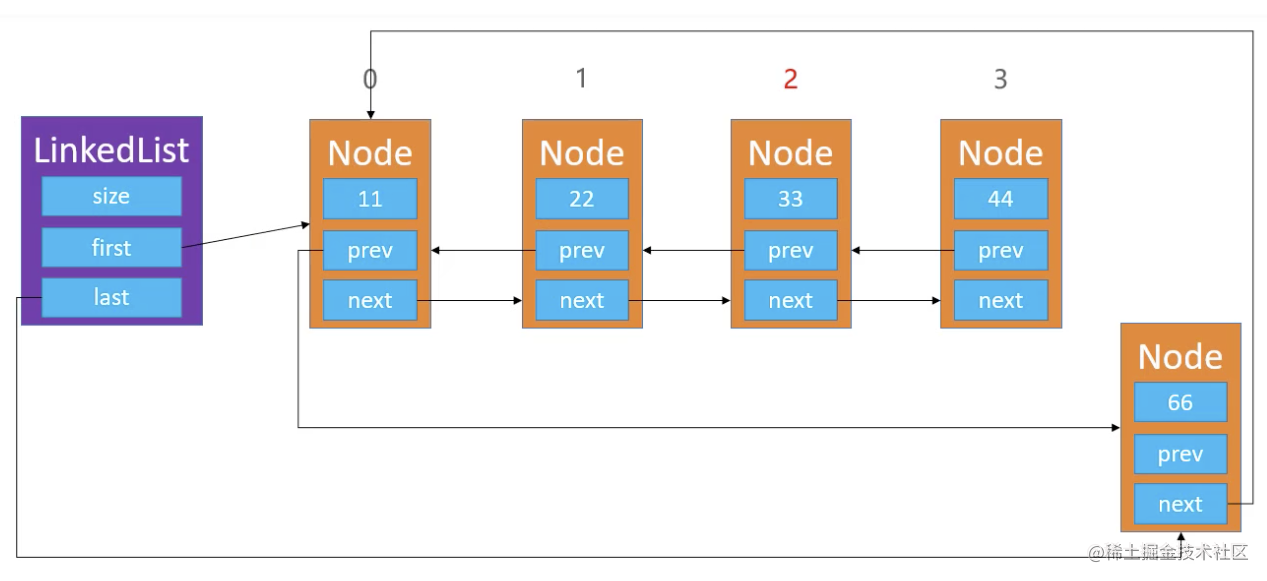#### 双向循环链表删除 - remove(int index, E element)

``` public E remove(int index) { rangeCheck(index);

``````Node<E> node = first;
if (size == 1) {
first = null;
last = null;
} else {
node = node(index);
Node<E> prev = node.prev;
Node<E> next = nodex.next;
prev.next = next;
next.prev = prev;

if (node == first) {// index == 0
first = next;
}
}

size--;
return node.element;
``````

} ```

### 约瑟夫问题(Jesephus Problem)

• 数到三开枪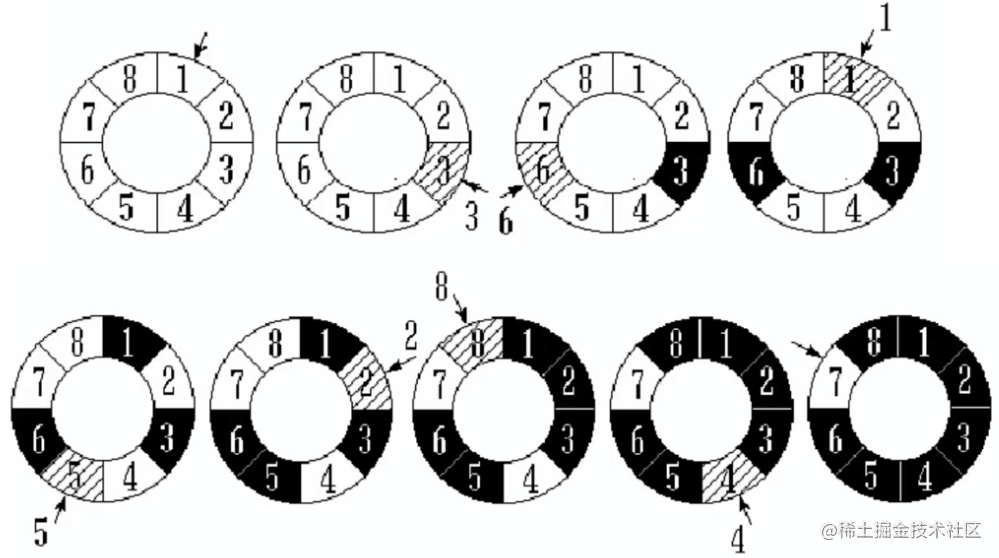• 可以考虑增设1个成员变量、3个方法
• current: 用于指向某个节点
• void reset(): 让current指向头结点`first`
• E next(): 让`current`往后走一步, 也就是`current = current.next`
• E remove(): 删除`current`指向的节点, 删除成功后让`current指向下一个节点`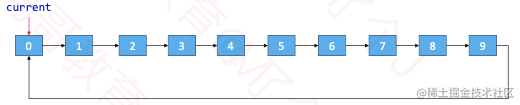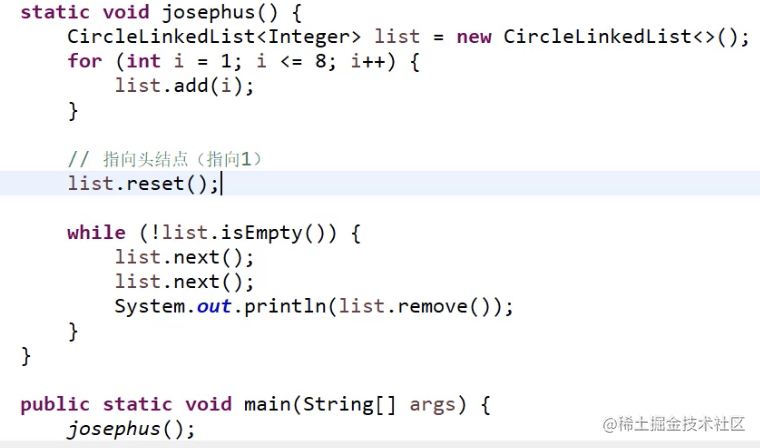### 静态链表

• 数组里面存放两个元素, 模拟链表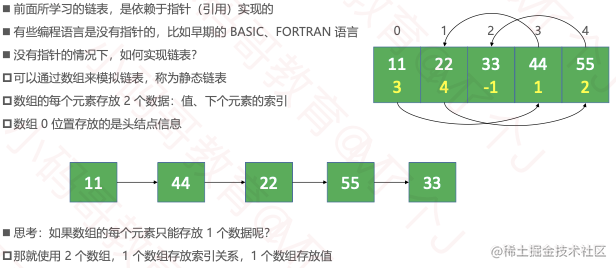### ArrayList能否进一步优化?

• int first: 存储首元素的位置
• 删除0号位, 改变first指针指向, first = 1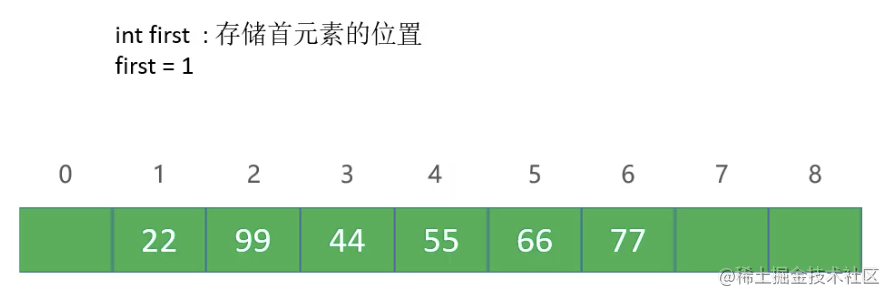### 双向链表和动态数组的区别

• 动态数组: 开辟、销毁内存空间的次数相对较少, 但可能造成内存空间浪费(可以通过缩容解决)
• 双向链表: 开启、销毁空间的次数相对较多, 但不会造成内存空间的浪费

• 如果频繁在`尾部`进行添加、删除操作, 动态数组、双向链表`均可`选择
• 如果频繁在`头部`进行添加、删除操作, 建议选择使用`双向链表`
• 如果有频繁的`在任意位置`添加、删除操作, 建议选择使用`双向链表`
• 如果有频繁的`查询`操作, 建议选择使用`动态数组`

# 二、树形结构, 树

## 树的基本概念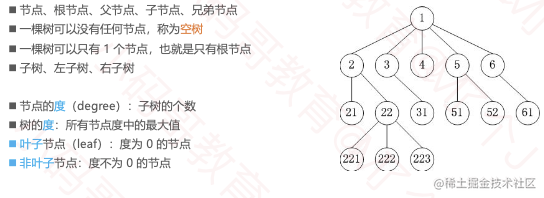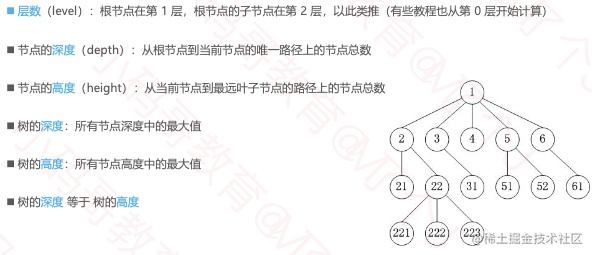## 二叉树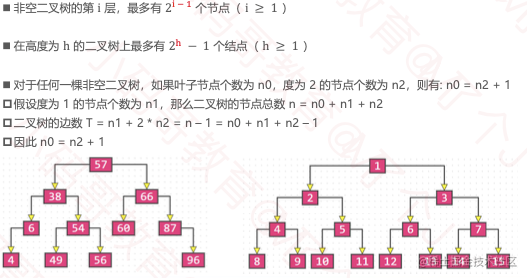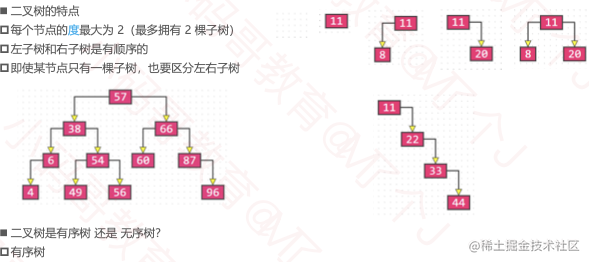• 二叉树的性质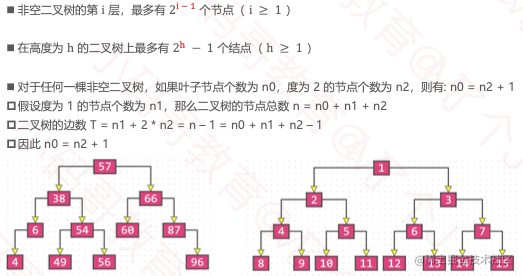### 真二叉树(Proper Binary Tree)

• 所有节点的度要么为0, 要么为2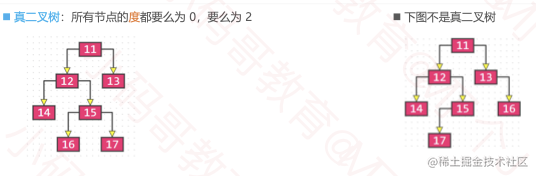### 满二叉树(Full Binary Tree)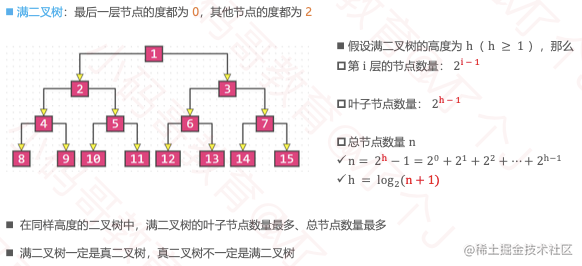### 完全二叉树(Complete Binary Tree)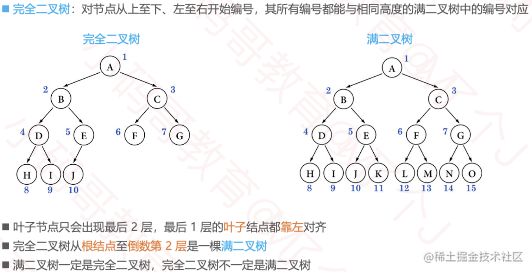- 完全二叉树的性质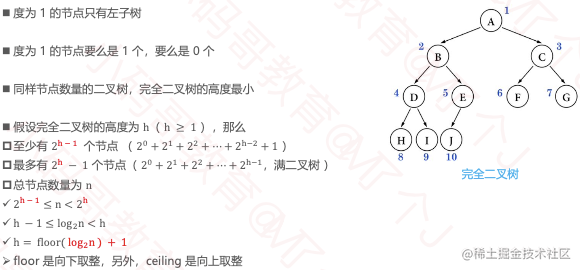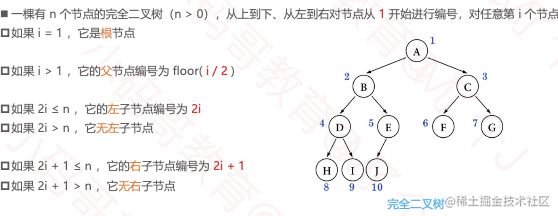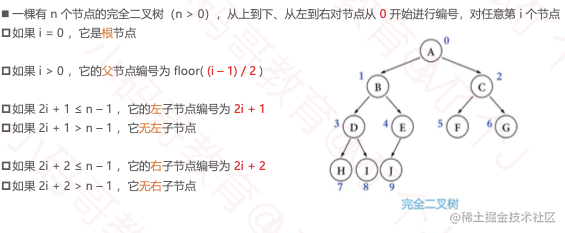• 下面就不是完全二叉树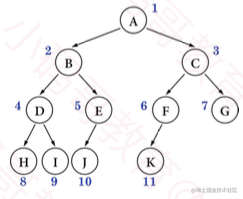### 常考点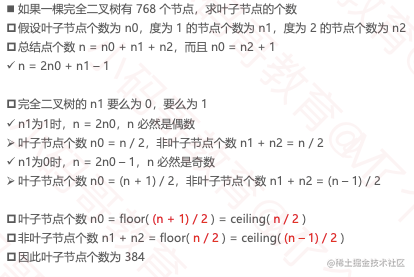### 二叉树的遍历

• 遍历是数据结构中的常见操作, 把所有元素都访问一遍
• 线性结构的遍历比较简单, 正序遍历/逆序遍历
• 根据节点访问顺序的也不同, 二叉树的常见遍历方式有四种
• 前序遍历
• 中序遍历
• 后序遍历
• 层序遍历

### 前序遍历(Preorder Traversal)

• 访问顺序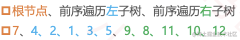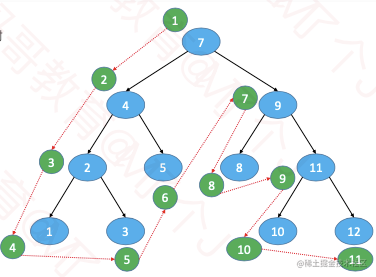### 中序遍历(Inorder Traversal)

• 访问顺序
• 中序遍历左子树、根节点、中序遍历右子树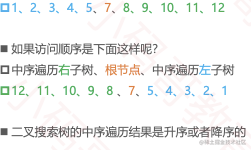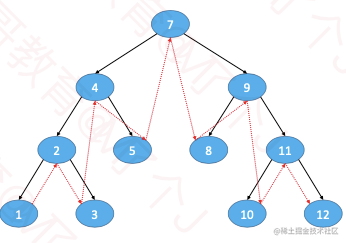### 后序遍历 (Postorder Traversal)

• 访问顺序
• 后序遍历左子树、后序遍历右子树、根节点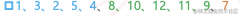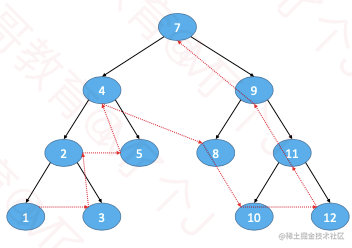### 层序遍历 (Level Order Traversal)

• 访问顺序
• 从上到下、从左到右依次访问每一个节点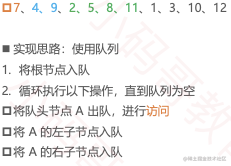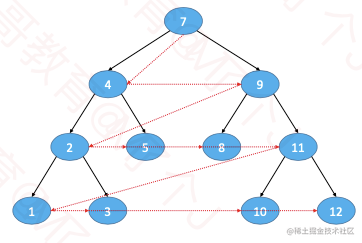### 力扣226. 翻转二叉树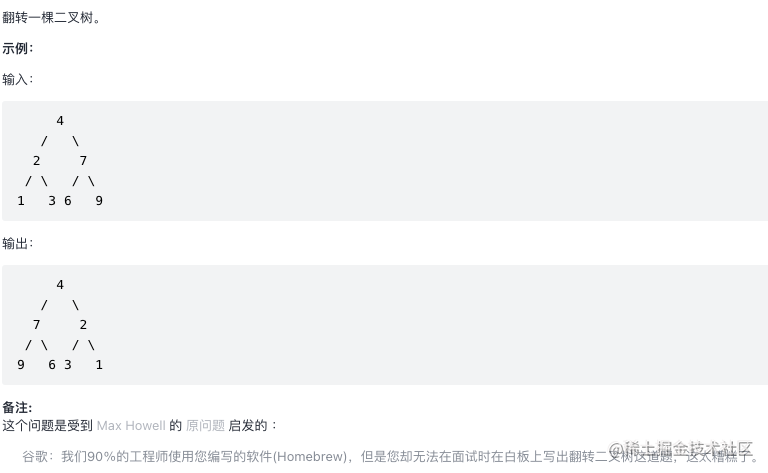``` /* * Definition for a binary tree node. * public class TreeNode { * int val; * TreeNode left; * TreeNode right; * TreeNode() {} * TreeNode(int val) { this.val = val; } * TreeNode(int val, TreeNode left, TreeNode right) { * this.val = val; * this.left = left; * this.right = right; * } * } / class Solution { public TreeNode invertTree(TreeNode root) { if (root == null) return null;

``````    // 前序遍历, 先自己, 再左右
TreeNode tmpNode = root.left;
root.left = root.right;
root.right = tmpNode;

invertTree(root.left);
invertTree(root.right);

return root;
}
``````

}

class Solution { public TreeNode invertTree(TreeNode root) { if (root == null) return null;

``````    // 后序遍历, 先左右, 再自己
invertTree(root.left);
invertTree(root.right);

TreeNode tmpNode = root.left;
root.left = root.right;
root.right = tmpNode;

return root;
}
``````

}

class Solution { public TreeNode invertTree(TreeNode root) { if (root == null) return null;

``````    // 中序遍历
invertTree(root.left);

TreeNode tmpNode = root.left;
root.left = root.right;
root.right = tmpNode;

invertTree(root.left);

return root;
}
``````

} ```

# 力扣刷题

## 排序

### 冒泡排序

• 执行流程
• 从头开始比较每一对相邻元素, 如果第一个比第二个大, 就交换他们的位置
• 执行完一轮后, 最末尾的那个元素就是最大的元素
• 忽略曾经找到的最大元素, 重复执行第一步, 直到全部元素有序
• 最坏、平均时间复杂度: O(n²)

• 最好时间复杂度: O(n)
• 空间复杂度: O(1) ``` for (int end = array.length - 1; end > 0; end--) { for (int begin = 1; begin <= end; begin++) { if (cmp(begin, begin - 1) < 0) { swap(begin, begin - 1); } } }

// OC写法 for (NSInteger end = result.count - 1; end > 0; end--) { for (NSInteger begin = 1; begin <= end; begin++) { NSInteger left = [result[begin-1] integerValue]; NSInteger right = [result[begin] integerValue]; if (left > right) { [result exchangeObjectAtIndex:begin-1 withObjectAtIndex:begin]; } } } `- 如果序列已经完全有序, 可以提前终止冒泡排序, 优化1` for (int end = array.length - 1; end > 0; end--) { boolean sorted = true; for (int begin = 1; begin <= end; begin++) { if (cmp(begin, begin - 1) < 0) { swap(begin, begin - 1); sorted = false; } } if (sorted) break; }

// OC写法 NSMutableArray *result = [[NSMutableArray alloc] initWithArray:@[@1, @2, @5, @4, @3]]; for (NSInteger end = result.count - 1; end > 0; end--) {     BOOL sorted = YES;     for (NSInteger begin = 1; begin <= end; begin++) {         NSInteger left = [result[begin-1] integerValue];         NSInteger right = [result[begin] integerValue];         if (left > right) {             [result exchangeObjectAtIndex:begin-1 withObjectAtIndex:begin]; sorted = NO;         }     }     if (sorted) {         break;     } } `- 如果序列尾部已经局部有序, 可以记录最后一次交换的位置, 减少比较次数, 优化2` for (int end = array.length - 1; end > 0; end--) { int sortedIndex = 1; for (int begin = 1; begin <= end; begin++) { if (cmp(begin, begin - 1) < 0) { swap(begin, begin - 1); sortedIndex = begin; } } end = sortedIndex; }

// OC写法 NSMutableArray *result = [[NSMutableArray alloc] initWithArray:@[@1, @2, @5, @4, @3]]; for (NSInteger end = result.count - 1; end > 0; end--) {     NSInteger sortedIndex = 1;     for (NSInteger begin = 1; begin <= end; begin++) {         NSInteger left = [result[begin-1] integerValue];         NSInteger right = [result[begin] integerValue];         if (left > right) {             [result exchangeObjectAtIndex:begin-1 withObjectAtIndex:begin];         }         sortedIndex = begin;     } end = sortedIndex; } ```

### 排序算法的稳定性(Stability)

• 如果相等的2个元素, 在排序前后的相对位置保持不变, 那么这是稳定的排序算法
• 排序前: 5, 1, 3𝑎, 4, 7, 3𝑏
• 稳定的排序: 1, 3𝑎, 3𝑏, 4, 5, 7
• 不稳定的排序:1, 3𝑏, 3𝑎, 4, 5, 7
• 对自定义对象进行排序时, 稳定性会影响最终的排序效果
• 冒泡排序属于稳定的排序算法
• 稍有不慎, 稳定的排序算法也能被写成不稳定的排序算法 ```for (NSInteger end = result.count - 1; end > 0; end--) { for (NSInteger begin = 1; begin <= end; begin++) { NSInteger left = [result[begin-1] integerValue]; NSInteger right = [result[begin] integerValue]; if (left >= right) {// > 不慎写成 >= [result exchangeObjectAtIndex:begin-1 withObjectAtIndex:begin]; } } }```

### 原地算法(In-place Algorithm)

• 什么是原地算法?
• 不依赖额外的资源或者依赖少数的额外资源, 仅依靠输出来覆盖输入
• 空间复杂度为O(1)的都可以认为是原地算法
• 非原地算法, 称为Not-in-place或者Out-of-place
• 冒泡排序属于In-place

## 选择排序

• 执行流程
• 从序列中找出最大的那个元素, 然后与最末尾的元素交换位置
• 执行完一轮后, 最末尾的那个元素就是最大的元素
• 忽略步骤1中已经找到的最大元素, 重复执行步骤1 ``` for (int end = array.length - 1; end > 0; end--) { int max = 0; for (int begin = 1; begin <= end; begin++) { if (cmp(max, begin) < 0) { max = begin; } } swap(max, end); }

// OC写法 NSMutableArray *result = [[NSMutableArray alloc] initWithArray:@[@1, @2, @5, @4, @3]]; for (NSInteger end = result.count - 1; end > 0; end--) {     NSInteger max = 0;     for (NSInteger begin = 1; begin <= end; begin++) {         NSInteger begin = [result[begin] integerValue];         NSInteger max = [result[max] integerValue];         if (begin > max) {             max = begin;         }     } [result exchangeObjectAtIndex:end withObjectAtIndex:max]; } ``` - 选择排序的交换次数要远少于冒泡排序, 平均性能优于冒泡排序 - 最好、最坏、平均时间复杂度: O(n²), 空间复杂度: O(1), 属于不稳定排序

## 堆排序

• 堆排序可以认为是对选择排序的一种优化.
• 大顶堆

## 数据结构&算法

### 掌握最常见的数据结构

• 数组 @[@"12", @"34"]
• 优点: 查询快index 遍历方便
• 缺点: 增删慢(找到第5个, 删掉, 678前移) 只能存储一种数据类型(新的tuple元组) 大小固定不方便扩容(int就固定了)
• 链表

• 优点: 增删快(改变链表的指针)
• 缺点: 查询特别麻烦(先从头结点依次走下去)
• 双向链表

• 指针指向前后数据
• @autoreleasepool
• 线性表

• 队列
• queue, 里面放任务
• 堆栈

• 栈, 压栈, 先进后出, 页面pop

### 树

• 二叉树, 遍历, 顺序, 二叉树的翻转
• 二叉树既有链表的好处, 也有数组的好处

### hash(散列表)

• 1-10 找到7, 遍历
• 二分法 减少了时间复杂度
• 一次到位, 直接通过key找到value, 字典的底层就是hash
• 哈希函数:f
• 1 - key - f(key) -> index=1
• 哈希 把值1放到index=1的位置,
• 11 12 13 15 18
• 浪费资源, 哈希函数没有设计好
• f(key) = key - 10 = index 定义域key > 10
• 计算简单 + 分布均匀, 直接地址法, 数据分析法,
• 平方取中法(增大落差范围, 导致冲突降低), 哈希冲突
• 取余法
• 9 13 14 15 16 18 % 10
• 9 3 4 5 6 8
• 数据分析 - 位运算 - index - 取值
• 设计出一个合理的, 分布均匀的哈希函数(散列函数)很难

#### 哈希冲突

• 平方取中法
• 9 13 14 15 16 18
• 81 169 196 225 256 324 -- 哈希冲突
• 继续哈希 - 再设计哈希函数
• 判断法 - 每一次移动
• 再平方法 - 减少你的操作
• 11 12 22 32
• 1 + 2^2 = 5
• 1 + 2^2 + 3^2 = 14
• 拉链法 -
• 11 12 22 32 42 52

### 常见的算法题目

#### 1. 字符串翻转

• Hello,word =>
• Dorw,olleh
• 两个变量记录, 一个从前面走, 一个从末尾走, 换到最中间为止
• 移动 换值 指针

```void char_reverse(char *cha) { // 定义第一个字符 // 空间的首位就是指针的位置 char *begin = cha; // 定义一个末尾 char *end = cha + strlen(cha) - 1; while (begin < end) { // 核心逻辑 -- 换值, 移动 char jh_tmp = *begin; *(begin++) = *end; *(end--) = jh_tmp; } }```

#### 2. 翻转链表

• 链表有个特性, 从头开始, 没有下标, 断开非常容易
• 建立一个空的头结点 ``` struct JHNode reverseList(struct JHNode head) { // 定义遍历指针, 初始化为头结点 struct JHNode p = head; // 反转后的链表头部 struct JHNode newH = NULL; // 遍历链表 while (p != NULL) { // 记录下一个节点 struct JHNode *temp = p->next; // 当前节点的next指向新链表头部 p->next = newH; // 更改新链表头部为当前节点 newH = p; // 移动p指针 p = temp; }

// 返回反转后的链表头结点 return newH; } ```

## 力扣刷题

### 力扣151. 翻转字符串里的单词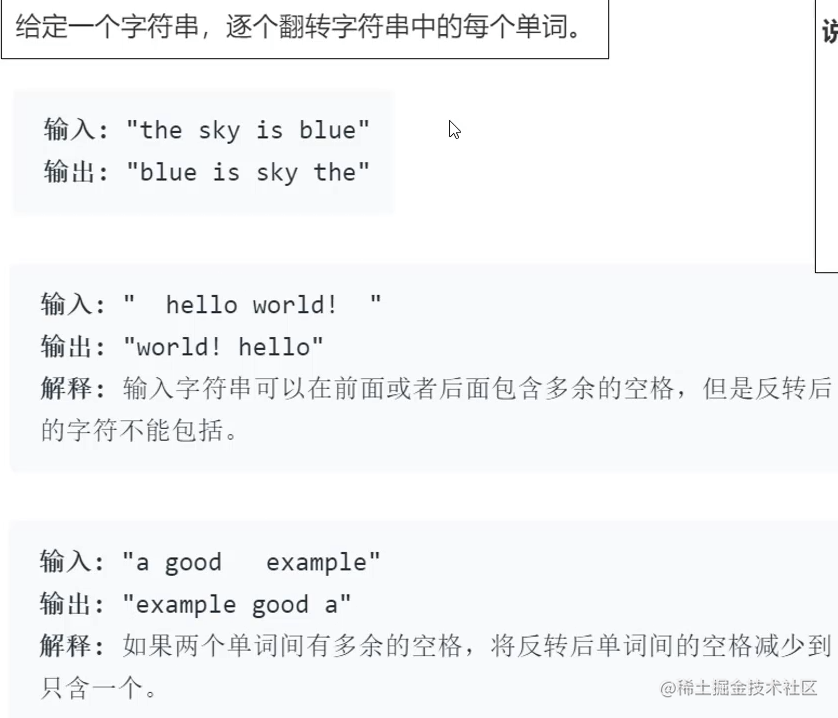1. 消除字符串中的多余空格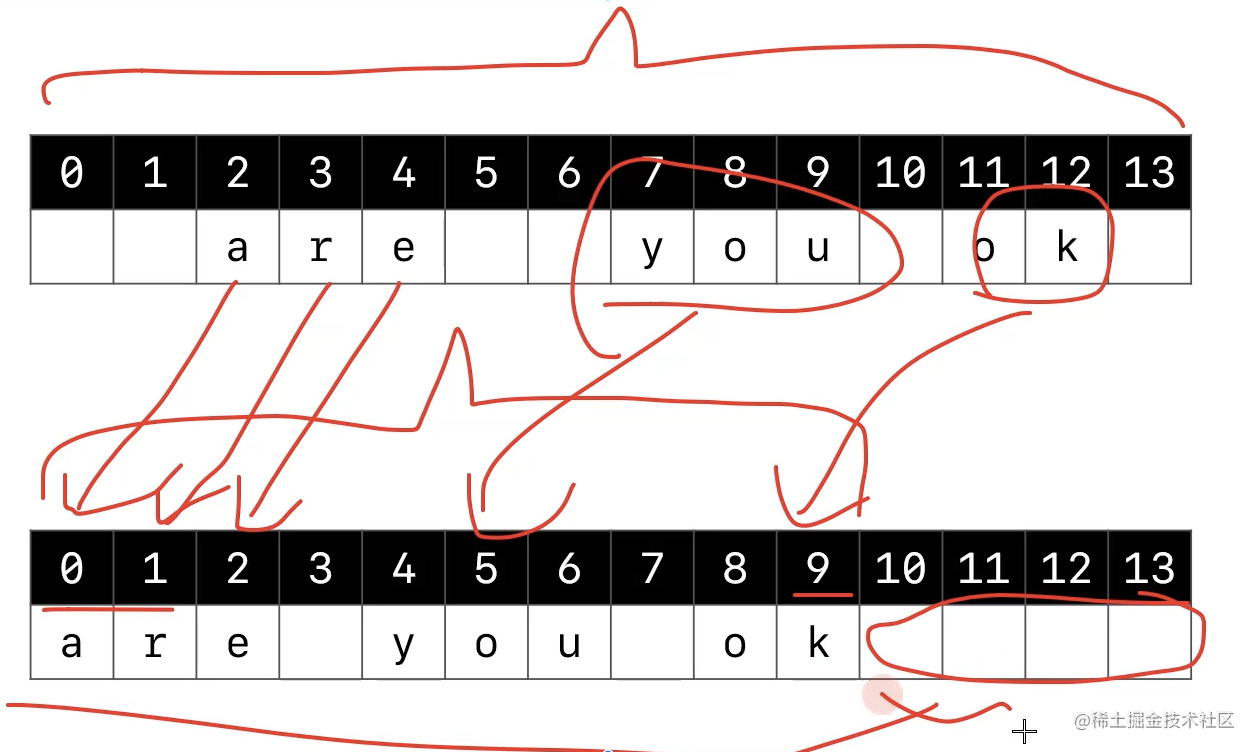2. 先0-10先逆序, 再逐个逆序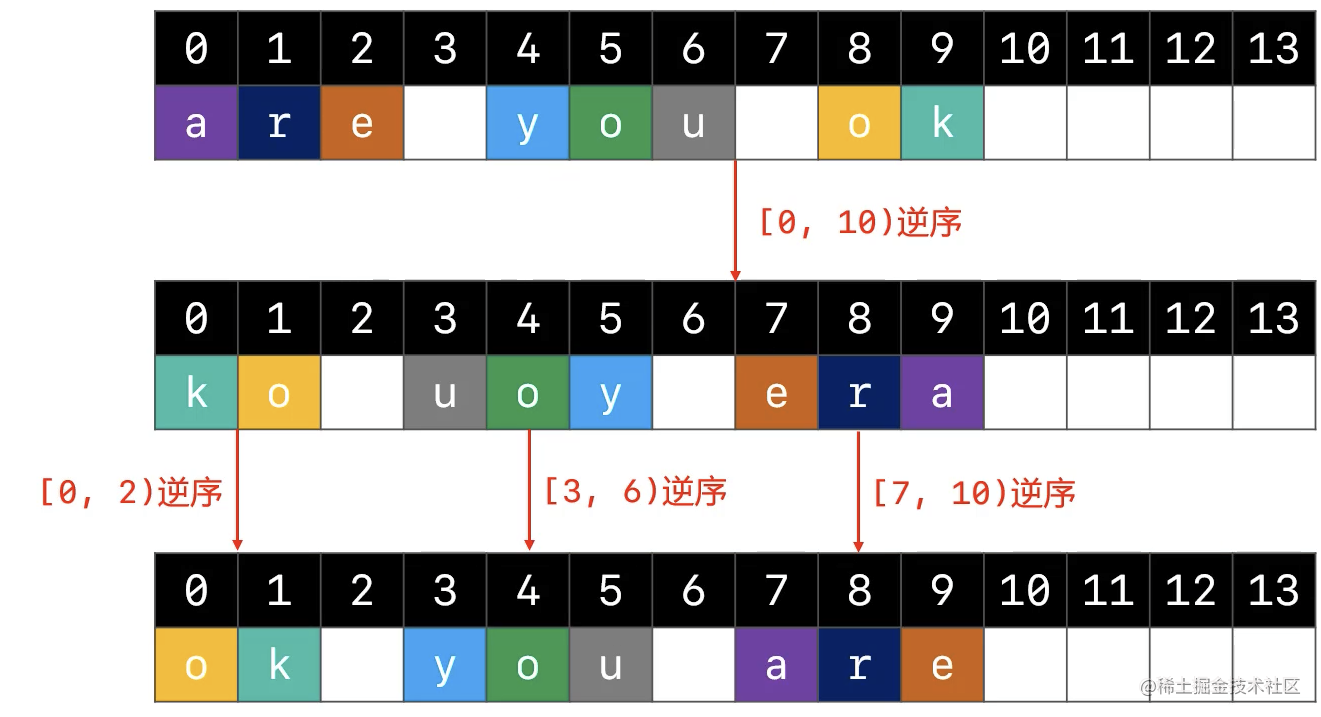``` public String reverseWords(String s) { // 容错处理 if (s == null) return ""; char[] chars = s.toCharArray();

``````    // 1. 清除多余的空格

// 字符串最终的有效长度
int len = 0;
// 当前用来存放字符的位置
int cur = 0;
// 前一个字符是为空格字符
boolean preIsSpace = true;
// 遍历字符串
for (int i = 0; i < chars.length; i++) {
if (chars[i] != ' ') {// 当前字符chars[i]不是空格字符
chars[cur] = chars[i];
cur++;
preIsSpace = false;// cur指针++移动后, 前一个字符不是空格字符
} else if (preIsSpace == false) {// chars[i]是空格字符 且 前一个字符chars[i - 1]不是空格
chars[cur] = ' ';
cur++;
preIsSpace = true;
}
}
// 遍历结束后, 最终的 前一个字符是空格字符
len = preIsSpace ? (cur - 1) : cur;
if (len <= 0) return "";

// 2. 对整个字符串进行逆序
reverse(chars, 0, len);

// 3. 对每一个单词进行逆序
// 前一个空格字符的位置(在-1位置有个假想的哨兵, 就是要一个假想的空格符)
int preSpaceIdx = -1;
for (int i = 0; i < len; i++) {
if (chars[i] != ' ') continue;
// 遍历到空格字符
reverse(chars, preSpaceIdx + 1, i);
preSpaceIdx = i;
}

// 4. 对最后一个单词进行逆序
reverse(chars, preSpaceIdx + 1, len);

return new String(chars, 0, len);
}

// 将[li, ri)范围内的字符串进行逆序
private void reverse(char[] chars, int li, int ri) {
ri--;
while (li < ri) {
char tmp = chars[li];
chars[li] = chars[ri];
chars[ri] = tmp;
li++;
ri--;
}
}
``````

} ```

### 力扣3. 无重复字符的最长子串

• 给定一个字符串, 请你找出其中不含有重复字符的最长子串的长度.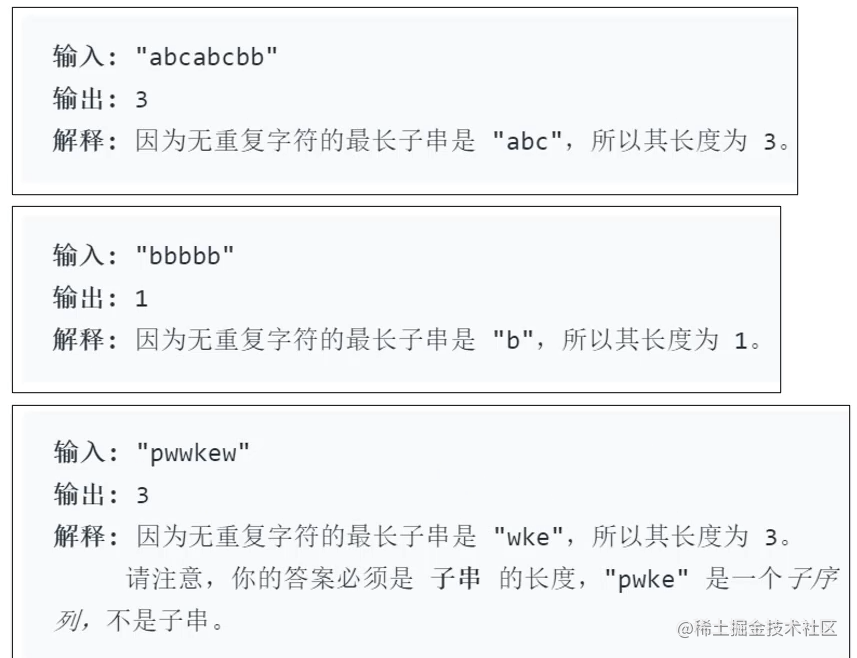• 有点动态规划的感觉

• 最长无重复字串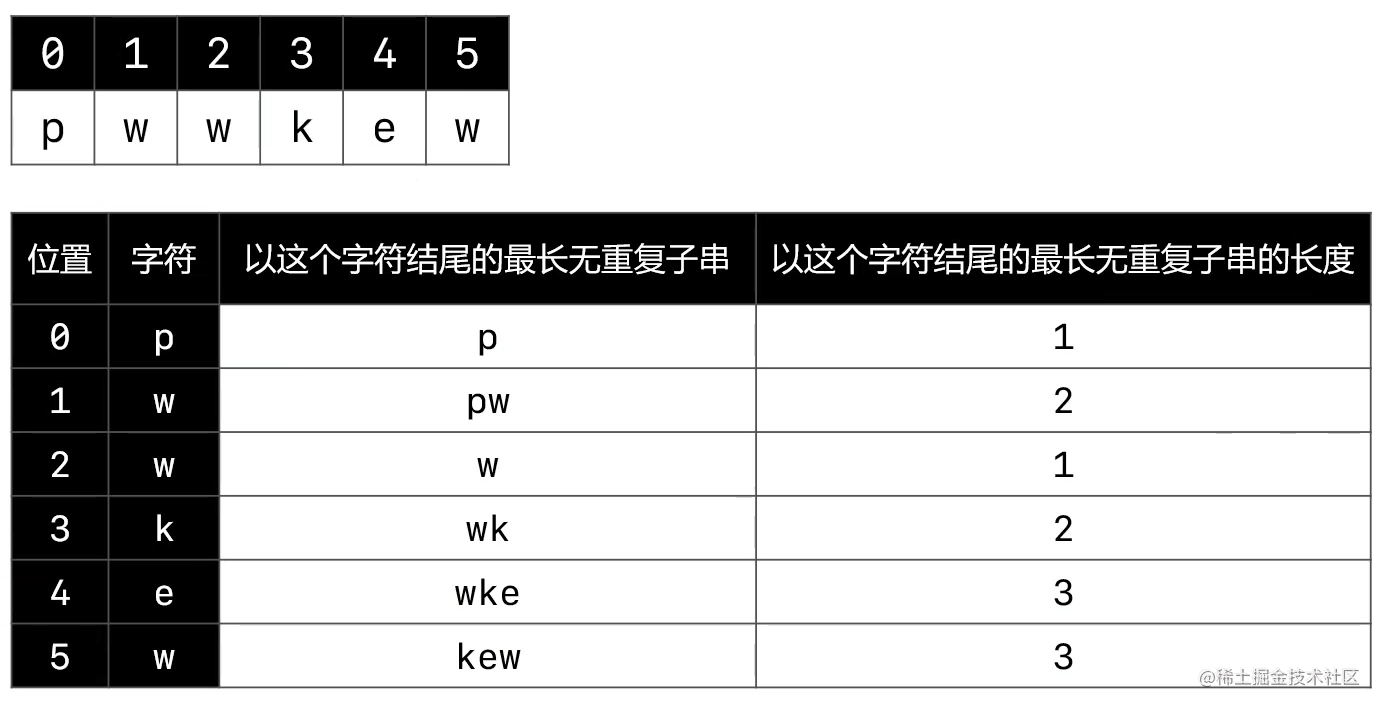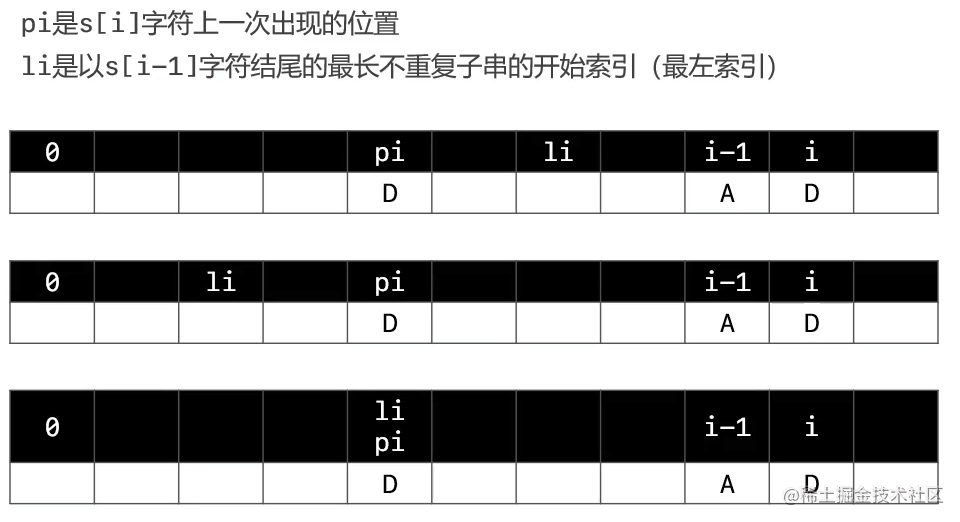- 哈希表技术 ``` public int lengthOfLongestSubstring(String s) { if (s == null) return 0; char[] chars = s.toCharArray(); if (chars.length== 0) return 0;

``````    // 用来保存每一个字符上一次出现的位置
Map<Character, Integer> prevIdxes = new HashMap<>();
// 扫描过零号位置
prevIdxes.put(chars, 0);

/**
// 小写字母26数组优化, ASCII 128, 假设是单字节字符
// 用来保存每一个字符上一次出现的位置
int[] prevIdxed = new int;
for (int i = 0; i < prevIdxed.length; i++) {
prevIdxes[i] = -1;
}
prevIdxes[chars] = 0;
*/

// 以i - 1位置字符结尾的最长不重复字符串的开始索引(最左索引)
int li = 0;
int max = 1;
for (int i = 1; i < chars.length; i++) {
// i位置字符上一次出现的位置
Integer pi = prevIdxes.get(chars[i]);
if (pi != null && li <= pi) {
li = pi +1;
}

// 存储这个字符出现的位置
prevIdxes.put(chars[i], i);

/**
// i位置字符上一次出现的位置
int pi = prevIdxes[chars[i]];
if (li <= pi) {
li = pi + 1;
}
// 存储这个字符出现的位置
prevIdxes[chars[i]] = i;
*/

// 求出最长不重复子串的长度
max = Math.max(max, i - li + 1);

}

return max;
}
``````

```

## 动态规划(Dynamic Programming)

• 动态规划, 建成DP
• 是求解最优化问题的一种常用策略
• 通常的使用套路(一步一步优化)
• 暴力递归(自顶向下, 出现了重叠子问题)
• 记忆化搜索(自顶向下)
• 递推(自底向上)

### 力扣剑指Offer47. 礼物的最大价值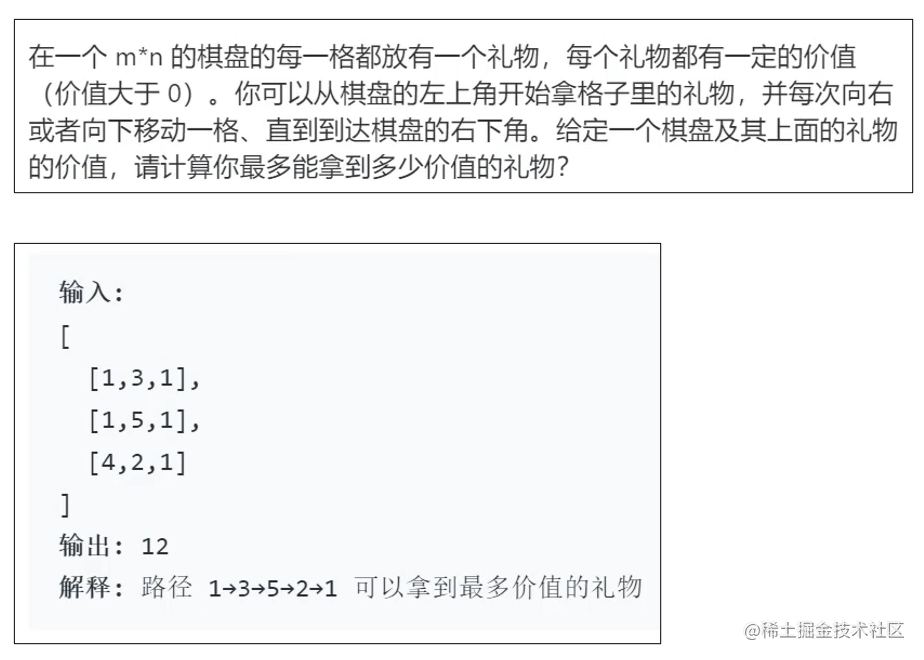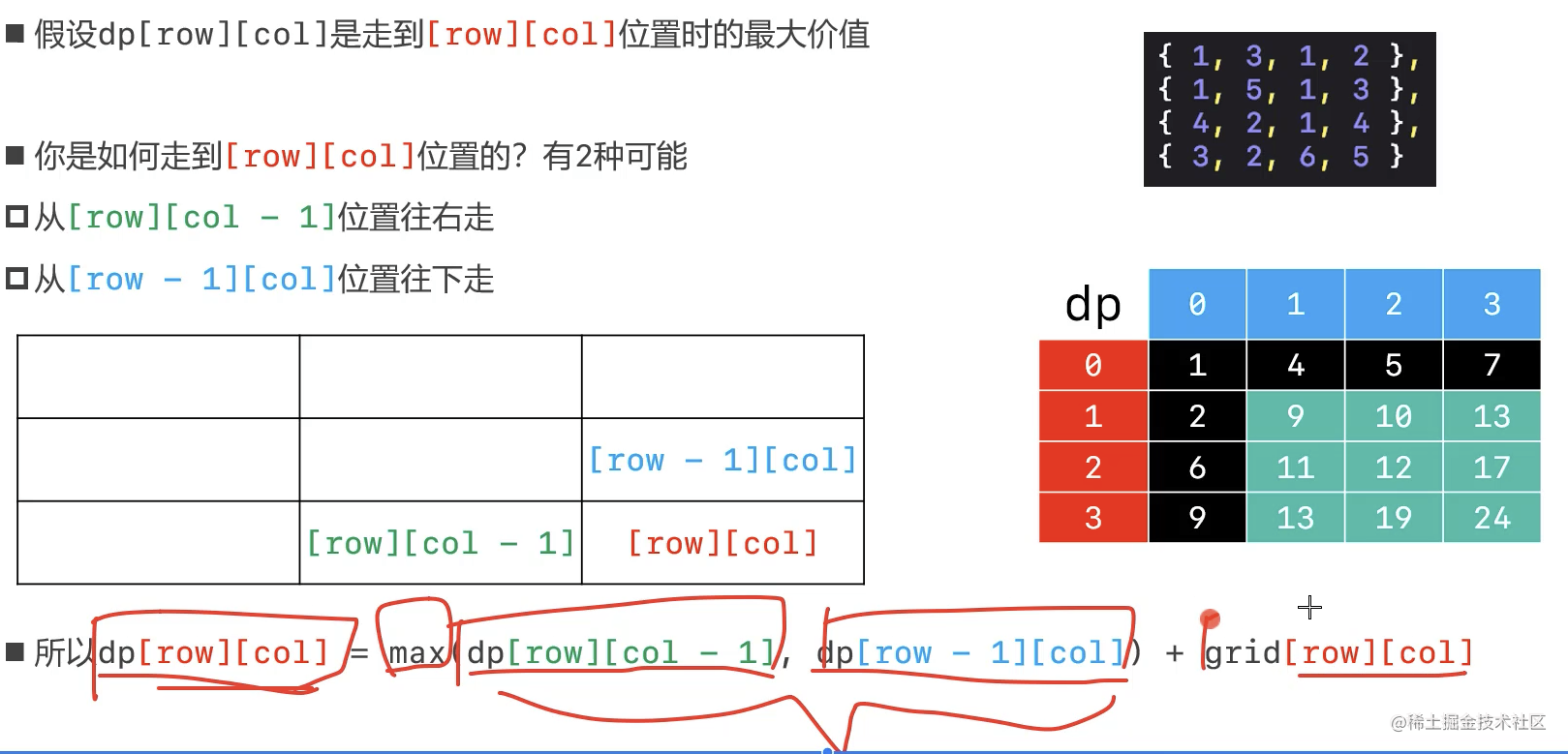``` public int maxValue(int[][] grid) { // 行数 int rows = grid.length; // 列数 int cols = grid.length;

``````    // 动态规划, 创建行列数的二维数组
int[][] dp = new int[rows][cols];

// 确定初始位置
dp = grid;

// 第0行, 遍历列, 给动态规划二维数组赋值0行全部列值
for (int col = 1; col < cols; col++) {
dp[col] = dp[col - 1] + grid[col];
}

// 第0列, 遍历行, 给动态规划二维数组赋值0列全部行值
for (int row = 1; row < rows; row++) {
dp[row] = dp[row - 1] + grid[row];
}

// 全部遍历, 对比哪个大, 赋值其他位置
for (int row = 1; row < rows; row++) {
for (int col = 1; col < cols; col++) {
dp[row][col] =  Math.max(dp[row - 1][col], dp[row][col - 1]) + grid[row][col];
}
}

return dp[rows - 1][cols - 1];
}
``````

```

### 力扣121. 买卖股票的最佳时机

• 给定一个数组 prices ，它的第 i 个元素 prices[i] 表示一支给定股票第 i 天的价格。
• 你只能选择 某一天 买入这只股票，并选择在 未来的某一个不同的日子 卖出该股票。设计一个算法来计算你所能获取的最大利润。
• 返回你可以从这笔交易中获取的最大利润。如果你不能获取任何利润，返回 0 。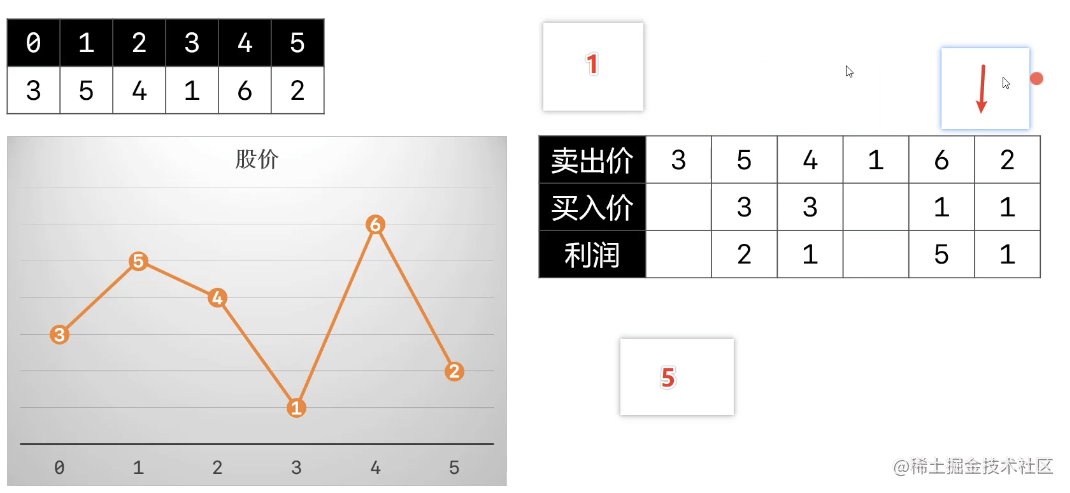``` public int maxProfit(int[] prices) { if ( prices == null || prices.length == 0) return 0;

``````// 当前扫描过的最小股票价格, 默认0号位
int minPrice = prices;
// 当前扫描过的最大利润
int maxProfit = 0;

// 从1号位开始遍历所有价格
for (int i = 1; i < prices.length; i++) {
if (prices[i] < minPrice) {// 最小股票价格小于第i天的价格
minPrice = prices[i];
} else {// 有最小股票价格时以第i天的价格卖出股票, 对比获得最大利润
maxProfit = Math.max(maxProfit, prices[i] - minPrice);
}
}

return maxProfit;
``````

} ```

### 力扣72. 编辑距离 困难

• 编辑距离算法被数据科学家广泛应用, 是用作机器翻译和语音识别评价标准的基本算法.
• 给定两个单词word1和word2, 计算出将word1转换成word2所使用的最少操作数.
• 你可以对一个单词进行如下三种操作:
• 插入一个字符
• 删除一个字符
• 替换一个字符 ```输入: word1 = "horse", word2 = "ros" 输出: 3 解释: horse -> rorse (将'h' 替换为 'r') rorse -> rose (删除 'r') rose -> ros (删除 'e')```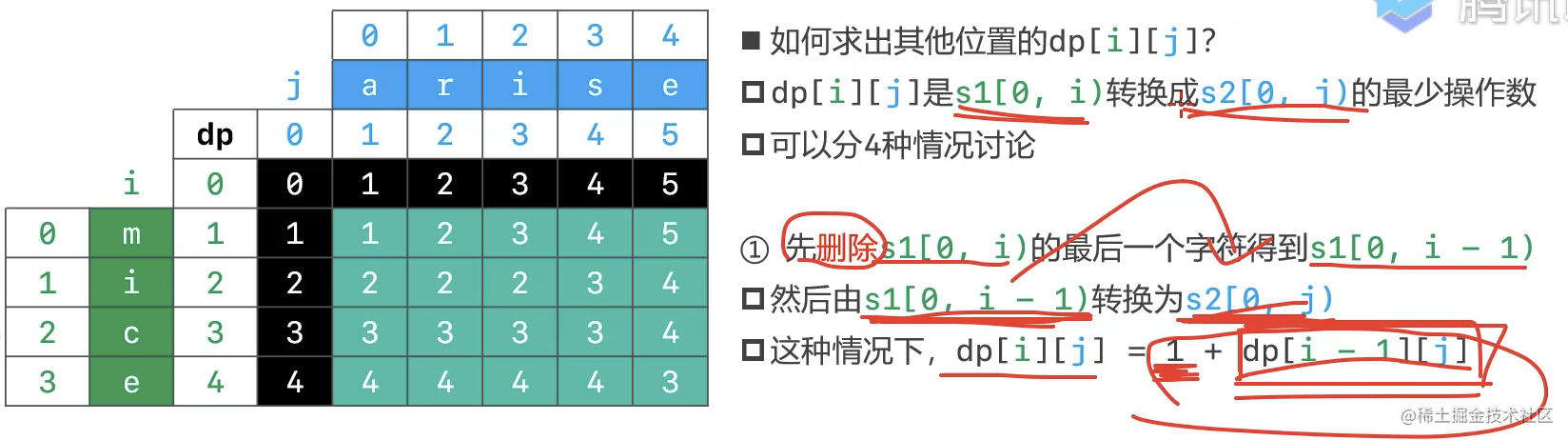• 前两种情况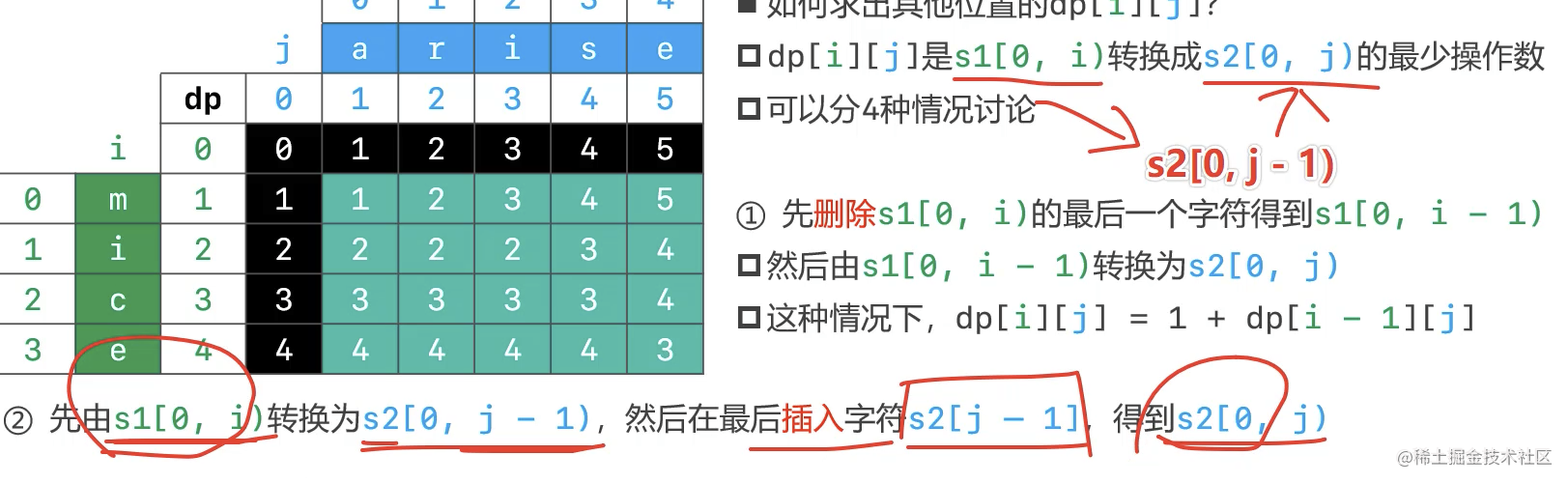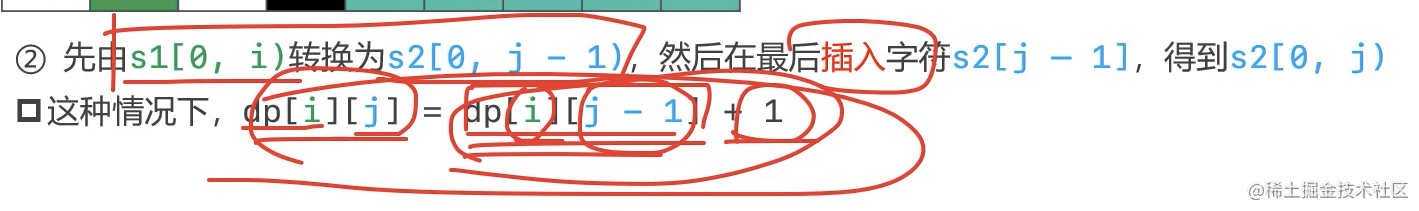• 第三种情况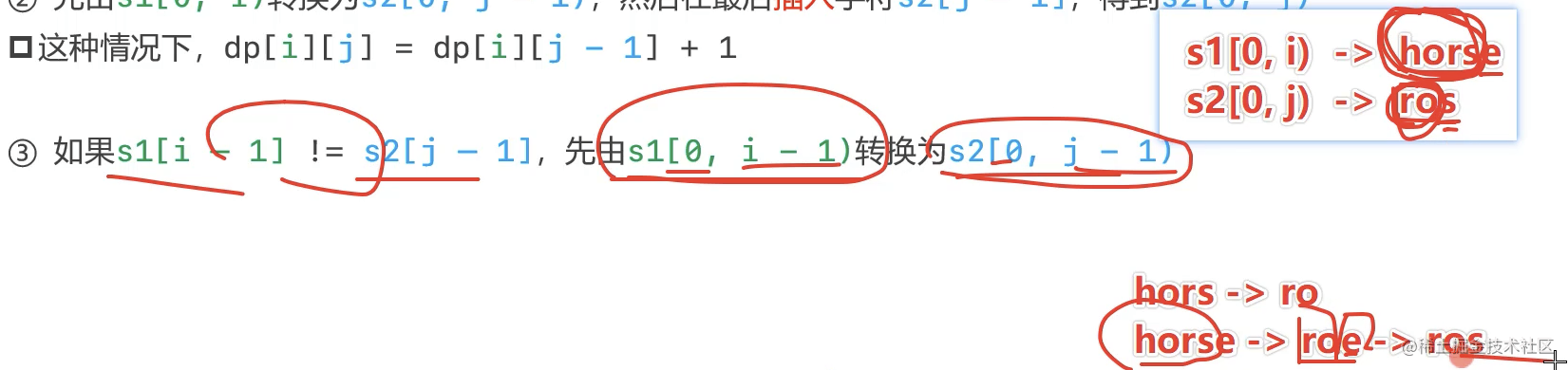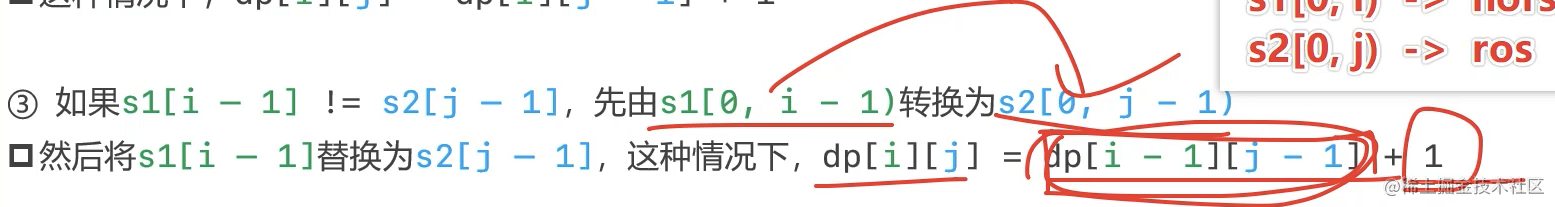• 第四种情况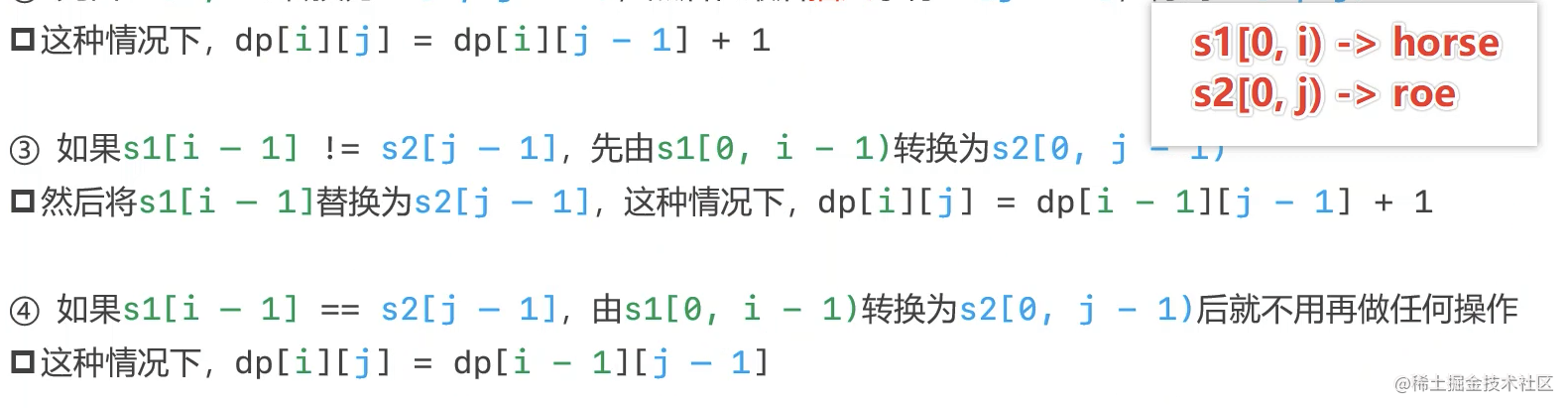• 三条路: 从上面、从左面、从左上角

• 挑一个最小的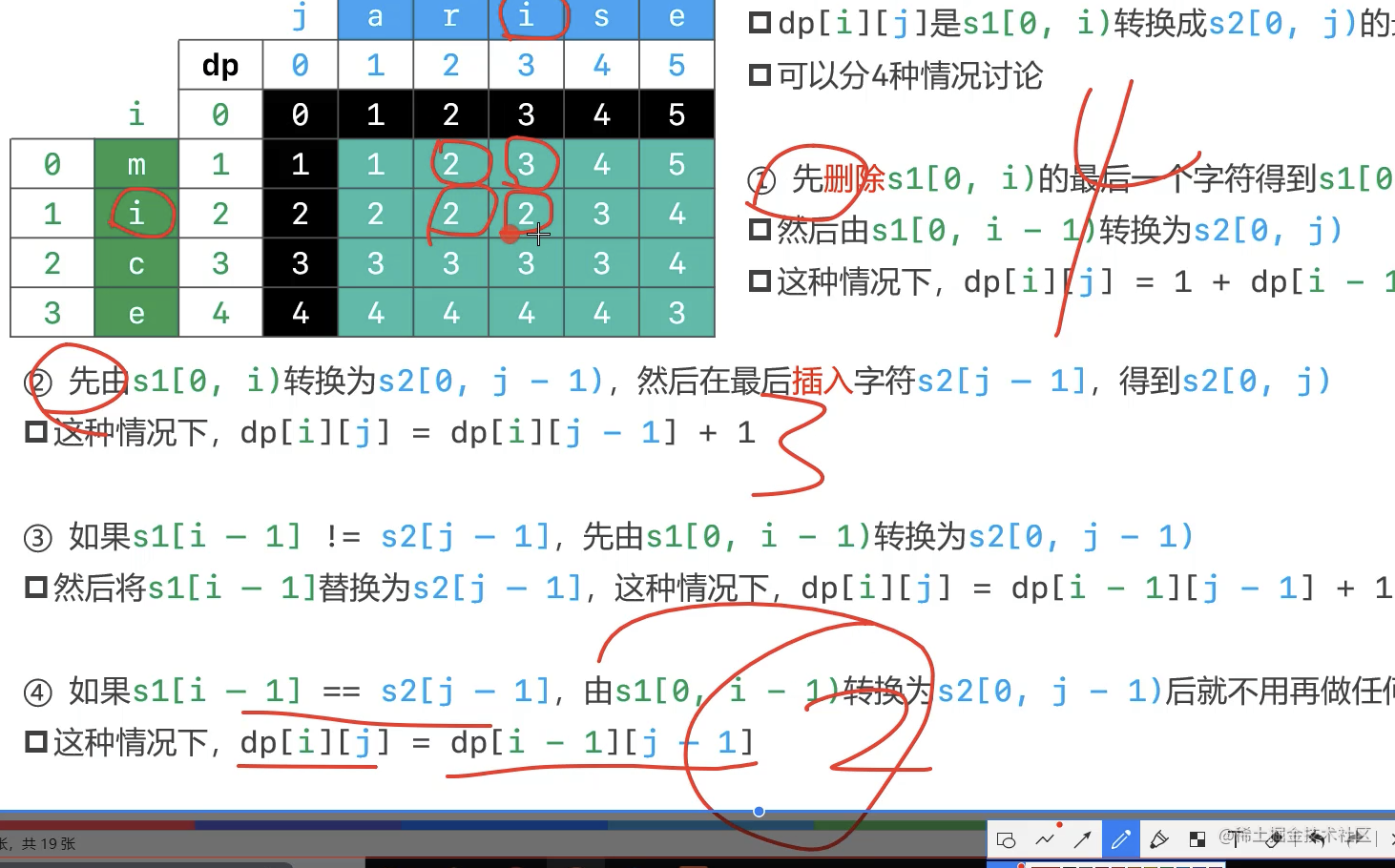``` public int minDistance(String word1, String word2) { if (word1 == null || word2 == null) return 0; char[] cs1 = word1.toCharArray();// 行 char[] cs2 = word2.toCharArray();// 列 int[][] dp = new int[cs1.length + 1][cs2.length + 1]; dp = 0;

``````    // 第0列, 最短路径就是当前行字符串的长度
for (int i = 1; i <= cs1.length; i++) {
dp[i] = i;
}

// 第0行, 最短路径就是当前列字符串的长度
for (int j = 1; j <= cs2.length; j++) {
dp[j] = j;
}

// 其他行其他列的最短路径
for (int i = 1; i <= cs1.length; i++) {
for (int j = 1; j <= cs2.length; j++) {
// 可能的三条计算路径: 从上面、从左面、从左上角
int top = dp[i - 1][j] + 1;// 上一行, 同一列
int left = dp[i][j - 1] + 1;// 上一列, 同一行
int leftTop = dp[i - 1][j - 1];

// 如果最后一个字符串不相等, 需要多一步替换操作
if (cs1[i - 1] != cs2[j - 1]) {
leftTop++;
}

dp[i][j] = Math.min(Math.min(top, left), leftTop);
}
}

return dp[cs1.length][cs2.length];
}
``````

``` - 难点在状态定义、状态转移方程, 怎么推导出下一个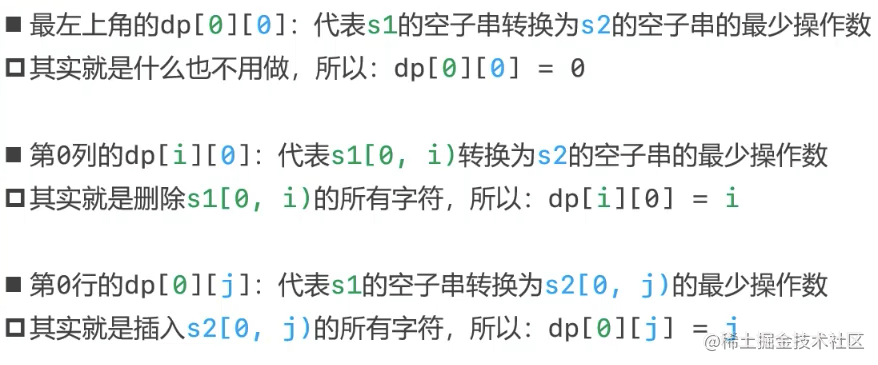• 二维数组可以优化成一维数组

### 力扣5. 最大回文子串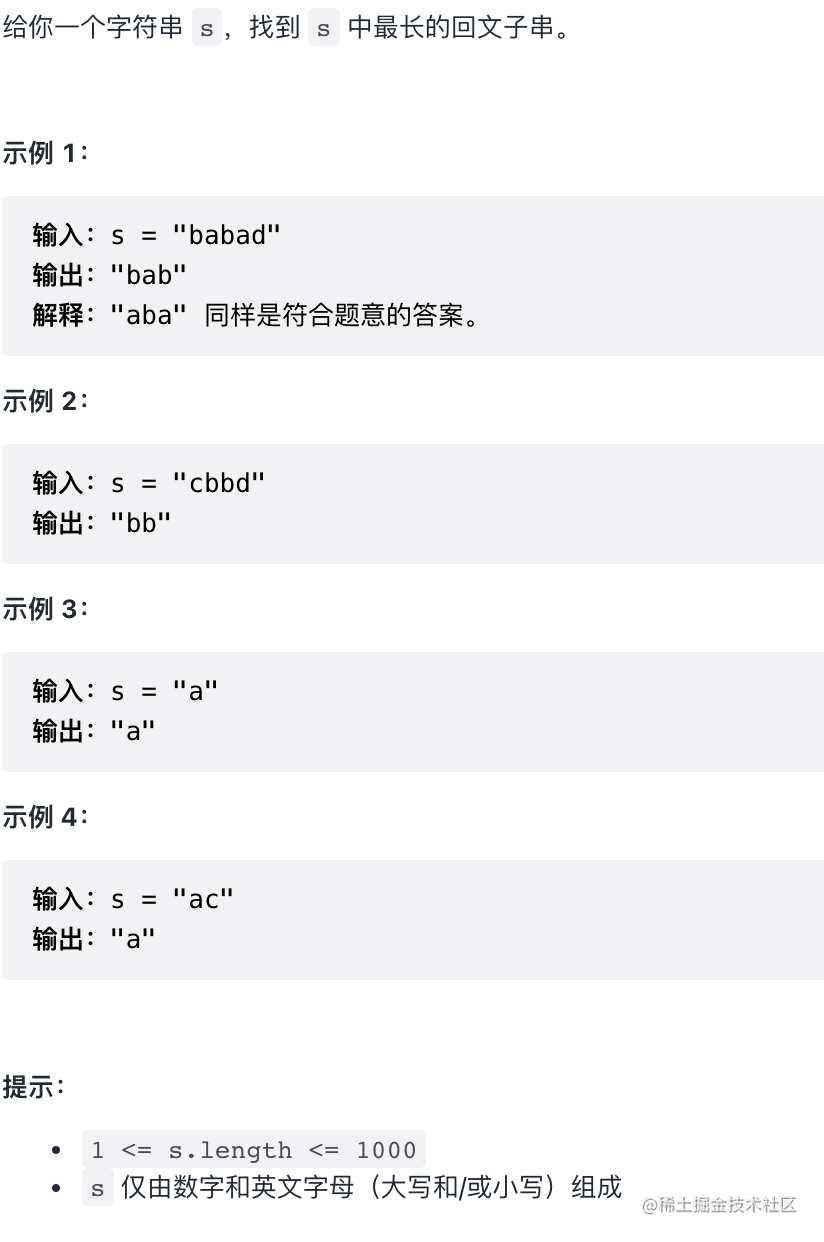#### 暴力法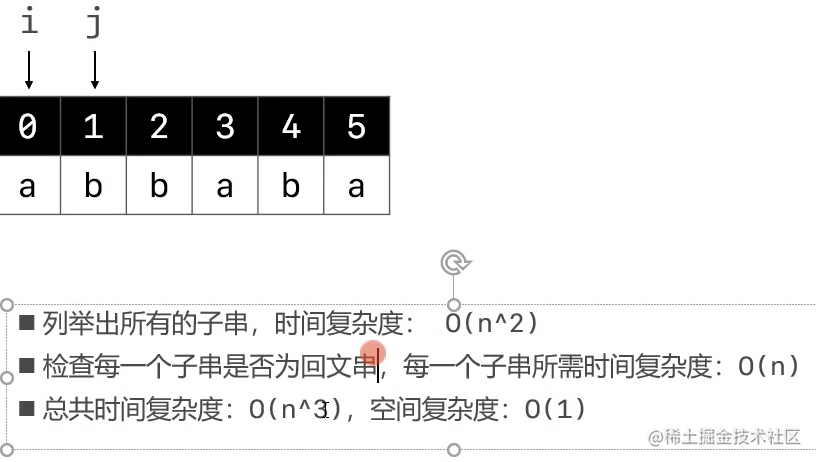#### 动态规划法

• 对比暴力法, 其实是暴力法的优化把时间复杂度优化从 n^3 到了 n^2
• 空间复杂度O(n^2), 空间换时间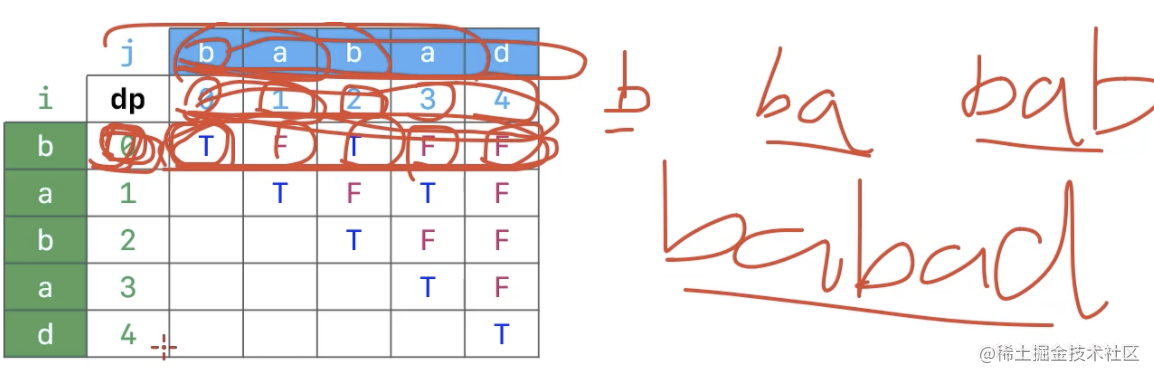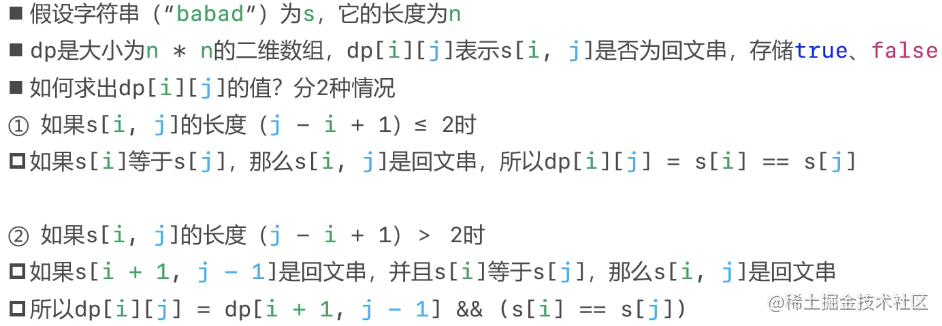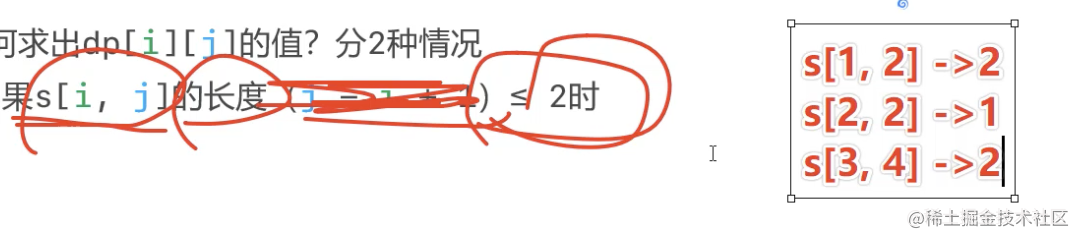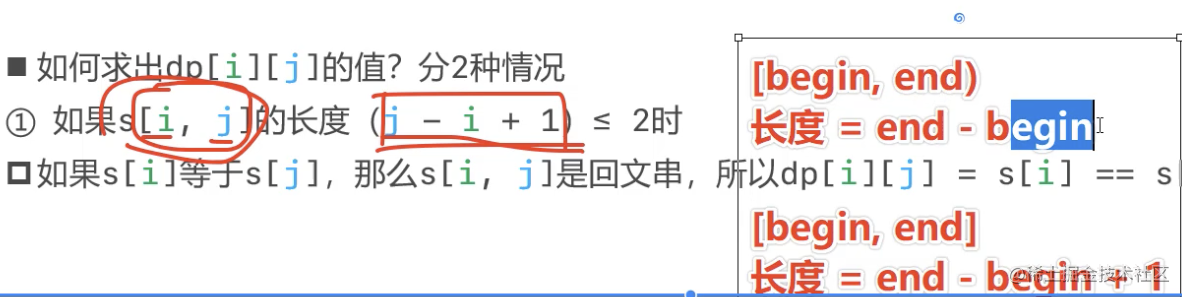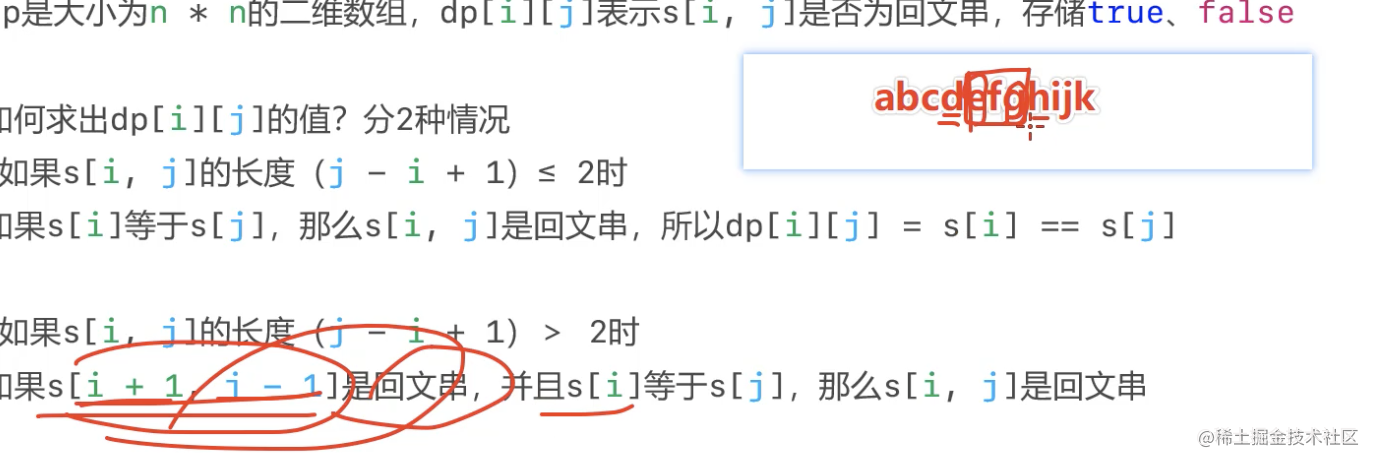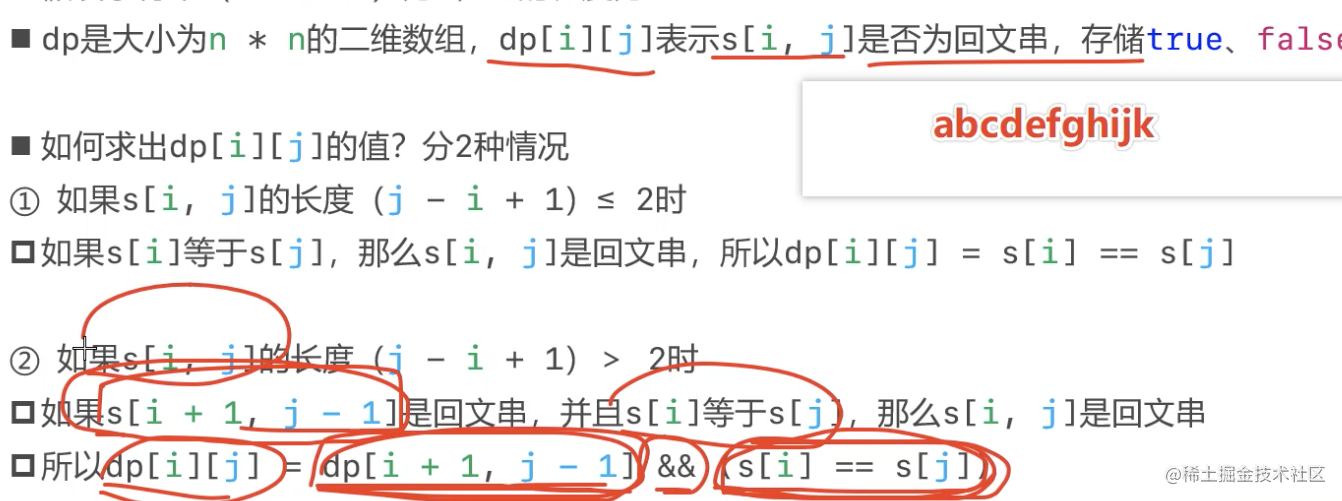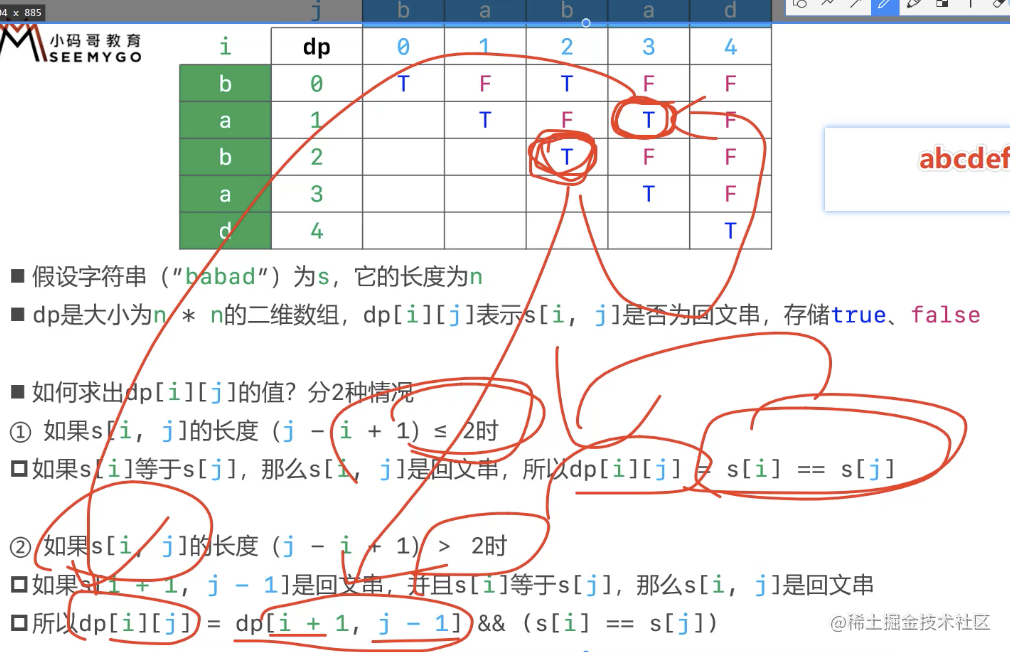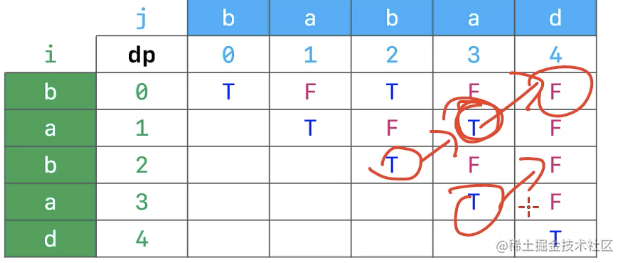- 从下到上, 从做到右 ``` public String longestPalindrome(String s) { if (s == null) return null; char[] cs = s.toCharArray(); if (cs.length == 0) return s;

``````    // 最长回文子串的长度(至少是1)
int maxLength = 1;
// 最长回文子串的开始索引
int begin = 0;

// 创建布尔类型的动态规划二维数组
boolean[][] dp = new boolean[cs.length][cs.length];

// 从下到上 (i由大到小)
for (int i = cs.length - 1; i >= 0; i--) {
// 从左到右(j有小到大)
for (int j = i; j < cs.length ; j++) {
// cs[i, j]字符串的长度
int length = j - i + 1;

// 两种情况
/**
1. 字符串长度 <= 2时, 长度为1或2, cs[i]字符等于cs[j]字符, 那么cs[i, j]是回文串,
此时dp[i][j] = cs[i] == cs[j]

2. 字符串长度 > 2时, 如果动态规划表当前字符串的左下方cs[i + 1, j - 1]是回文串 aaadefed,
且cs[i]字符等于cs[j]字符, 那么cs[i, j]是回文串
此时dp[i][j] = dp[i + 1][j - 1] && ()cs[i] == cs[j])
*/
dp[i][j] = (cs[i] == cs[j]) && (length <= 2 || dp[i + 1][j - 1]);

// 当前cs[i, j]是回文子串 且 长度大于保存的最大长度, 重新赋值
if (dp[i][j] && (length > maxLength)) {
maxLength = length;
begin = i;
}
}
}
return new String(cs, begin, maxLength);
}
``````

```

### 给定一个三角形 `triangle`, 找出自顶向下的最小路径和.

• 每一步只能移动到下一行中相邻的结点上. 相邻的结点 在这里指的是 下标上一层结点下标 相同或者等于 上一层结点下标 + 1 的两个结点. ```输入: triangle = [, [3,4], [6,5,7], [4,1,8,3]] 输出: 11 解释: 如下面简图所示```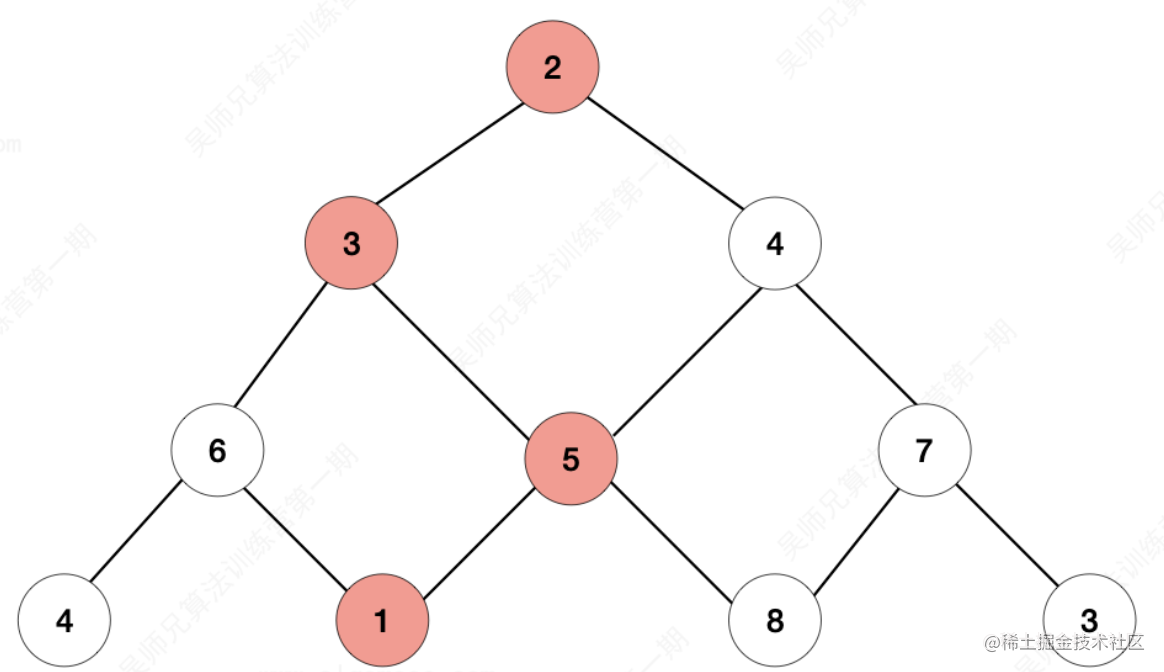- 自顶向下的最小路径和为11(即, 2 + 3 + 5 + 1 = 11). - 解法采用: 1. 从上往下的动态规划 2. 从下往上的动态规划 3. 从下往上的动态规划(使用一维数组)

• 思路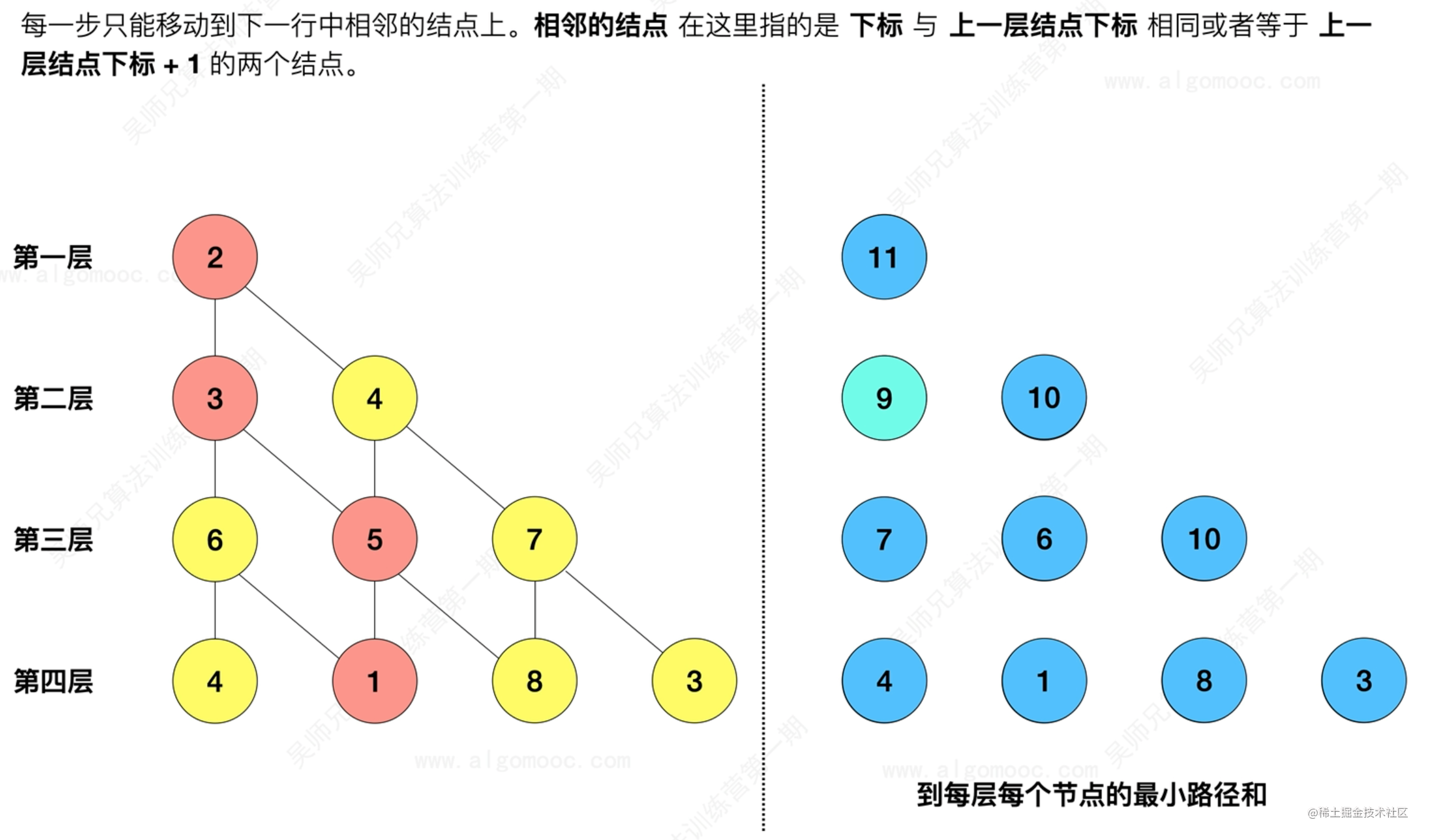• 存储的是到达第i+1层各个结点的最小路径之和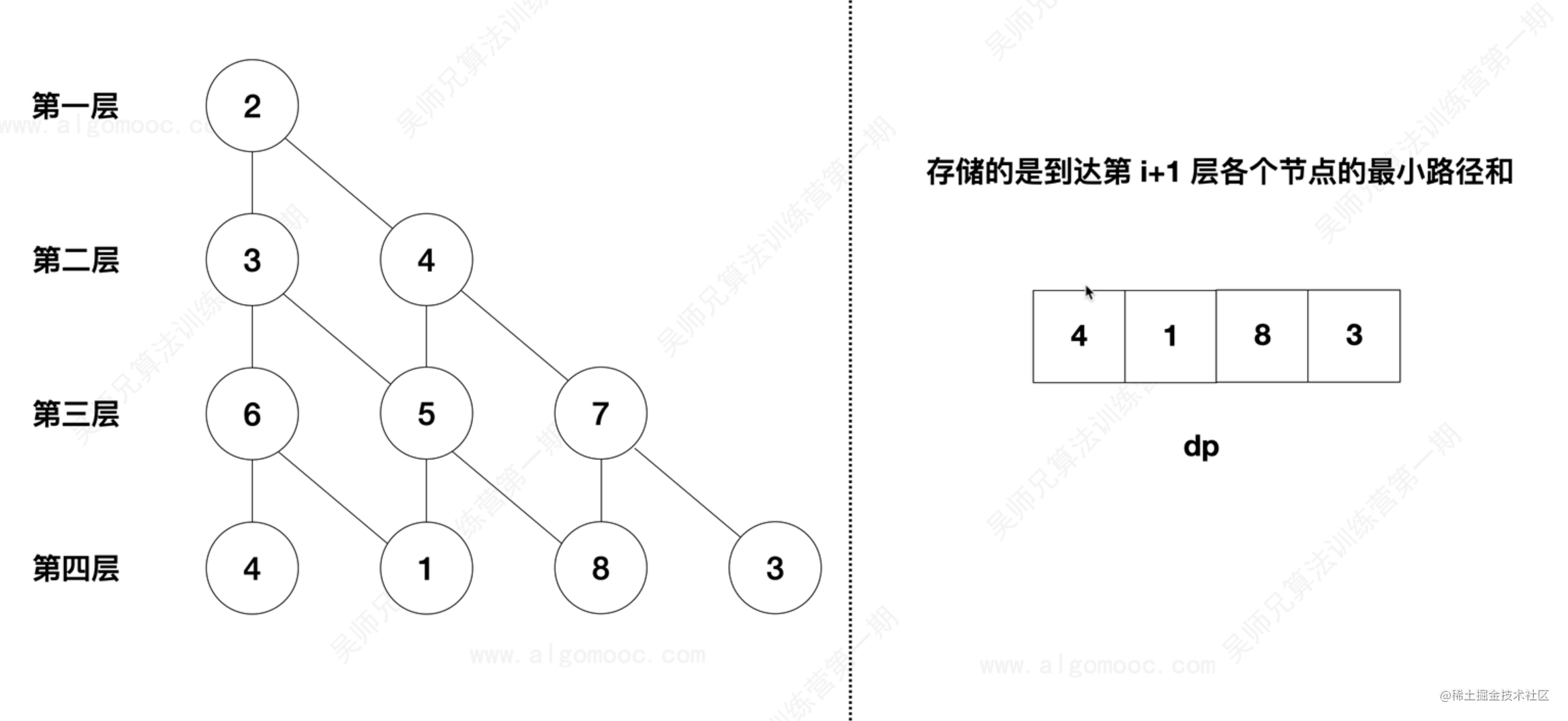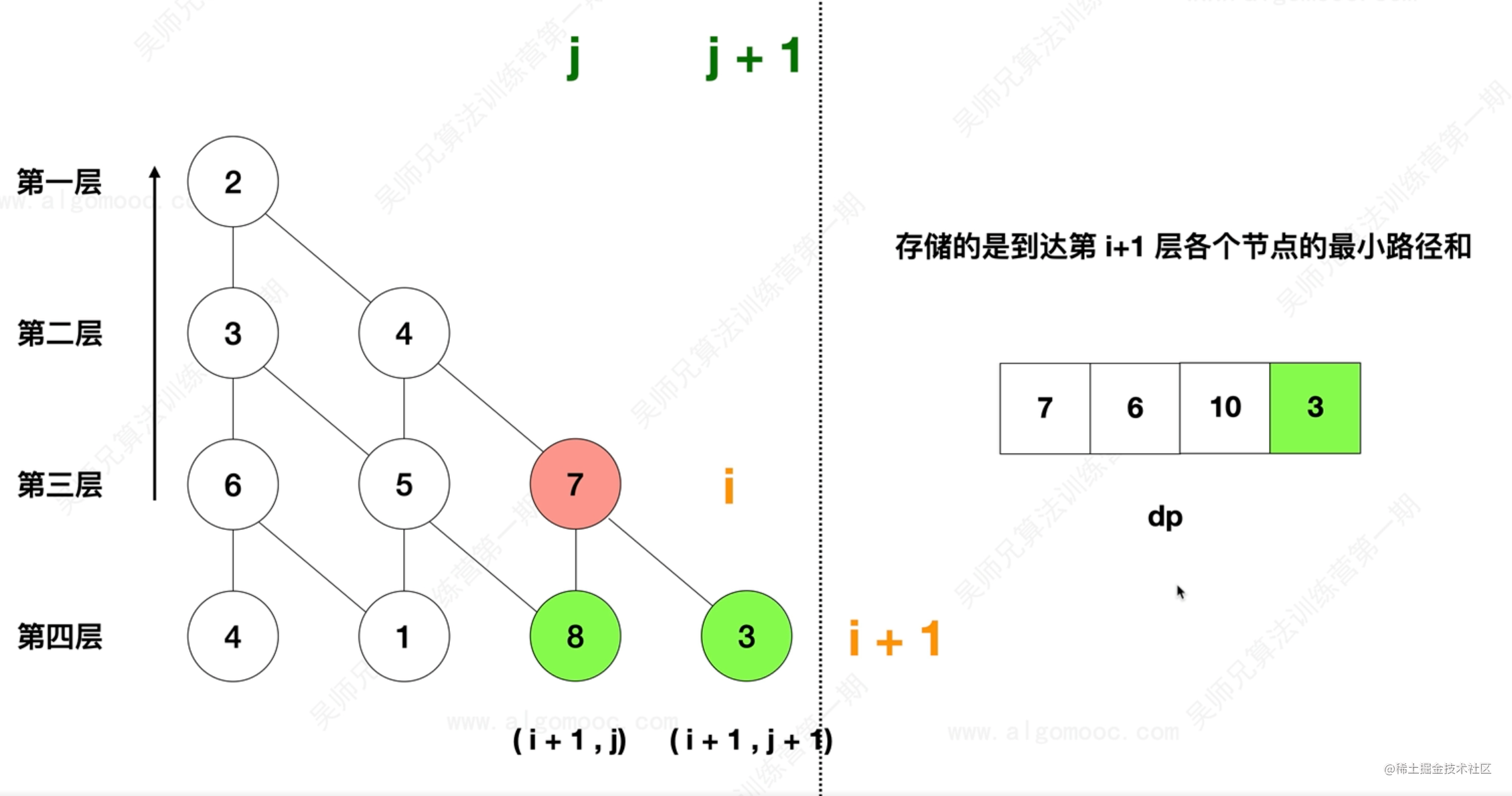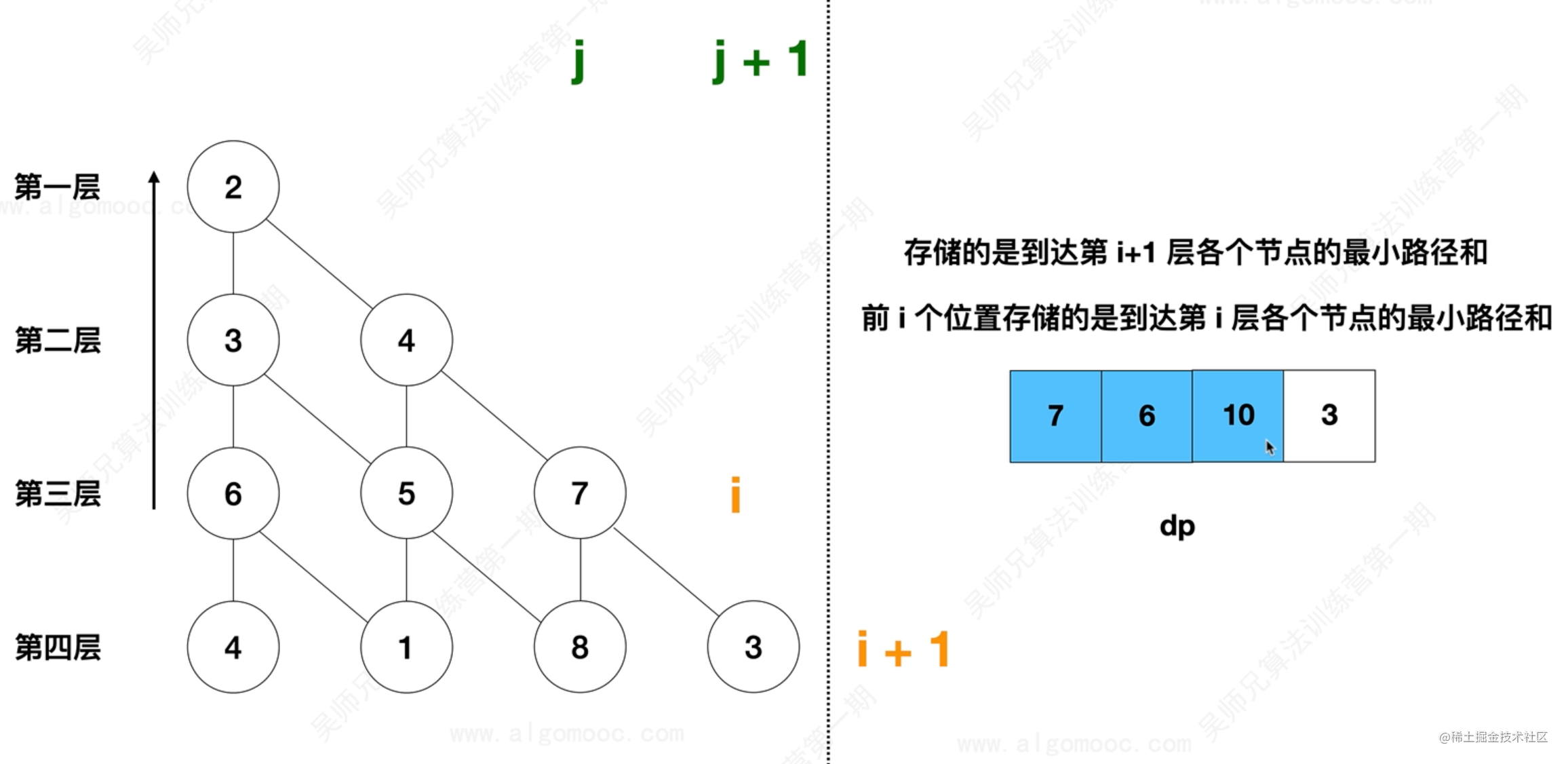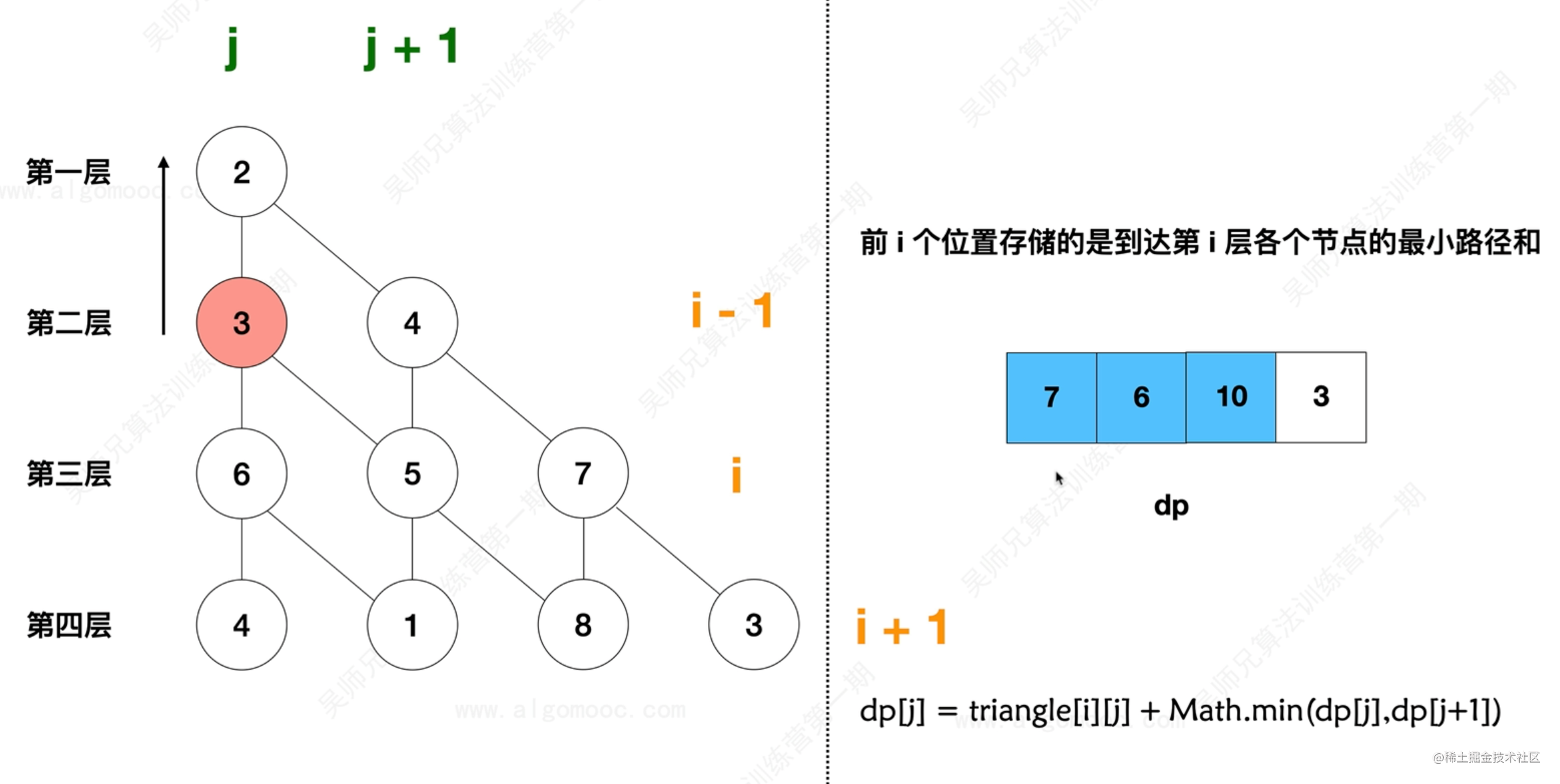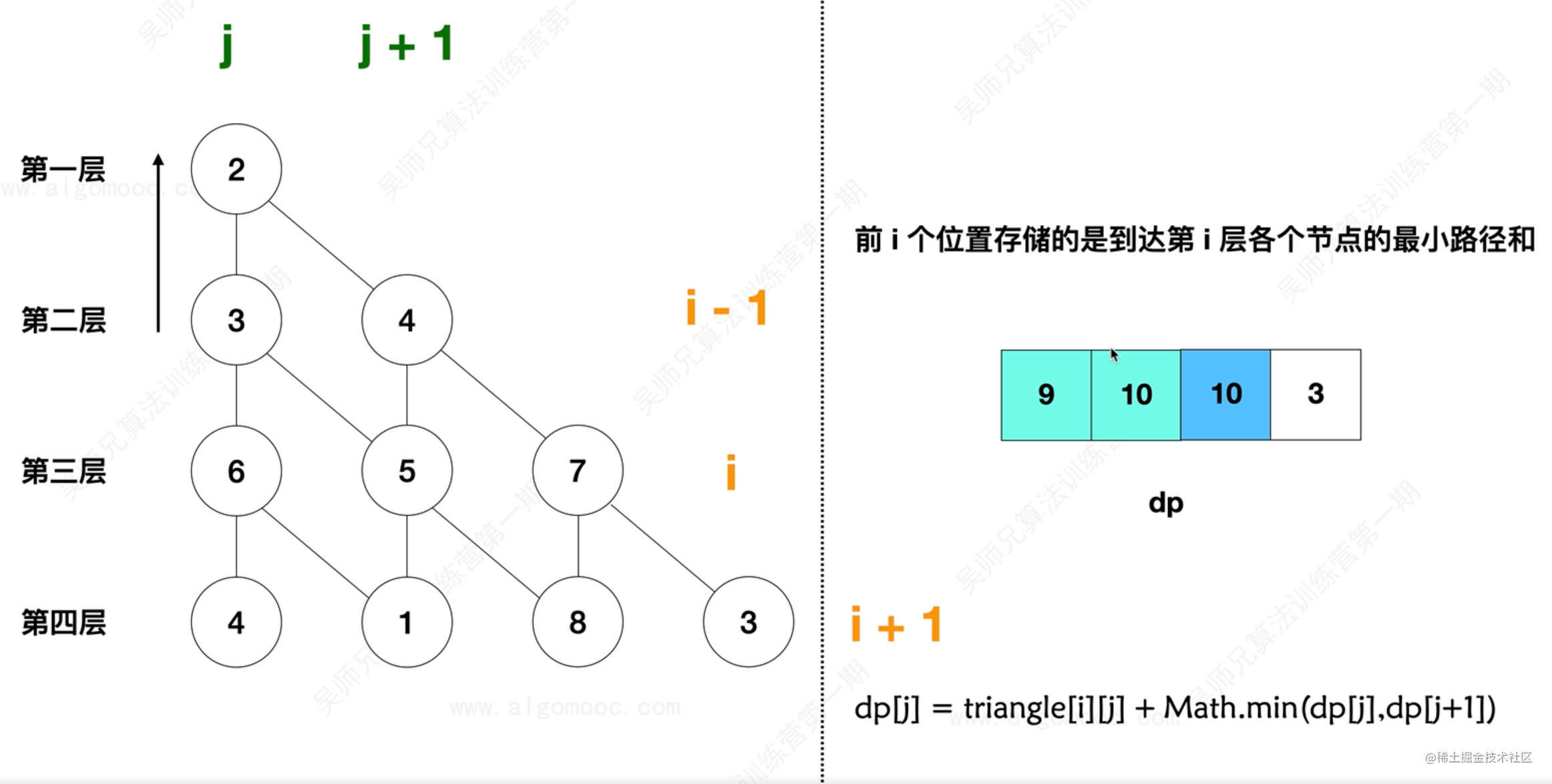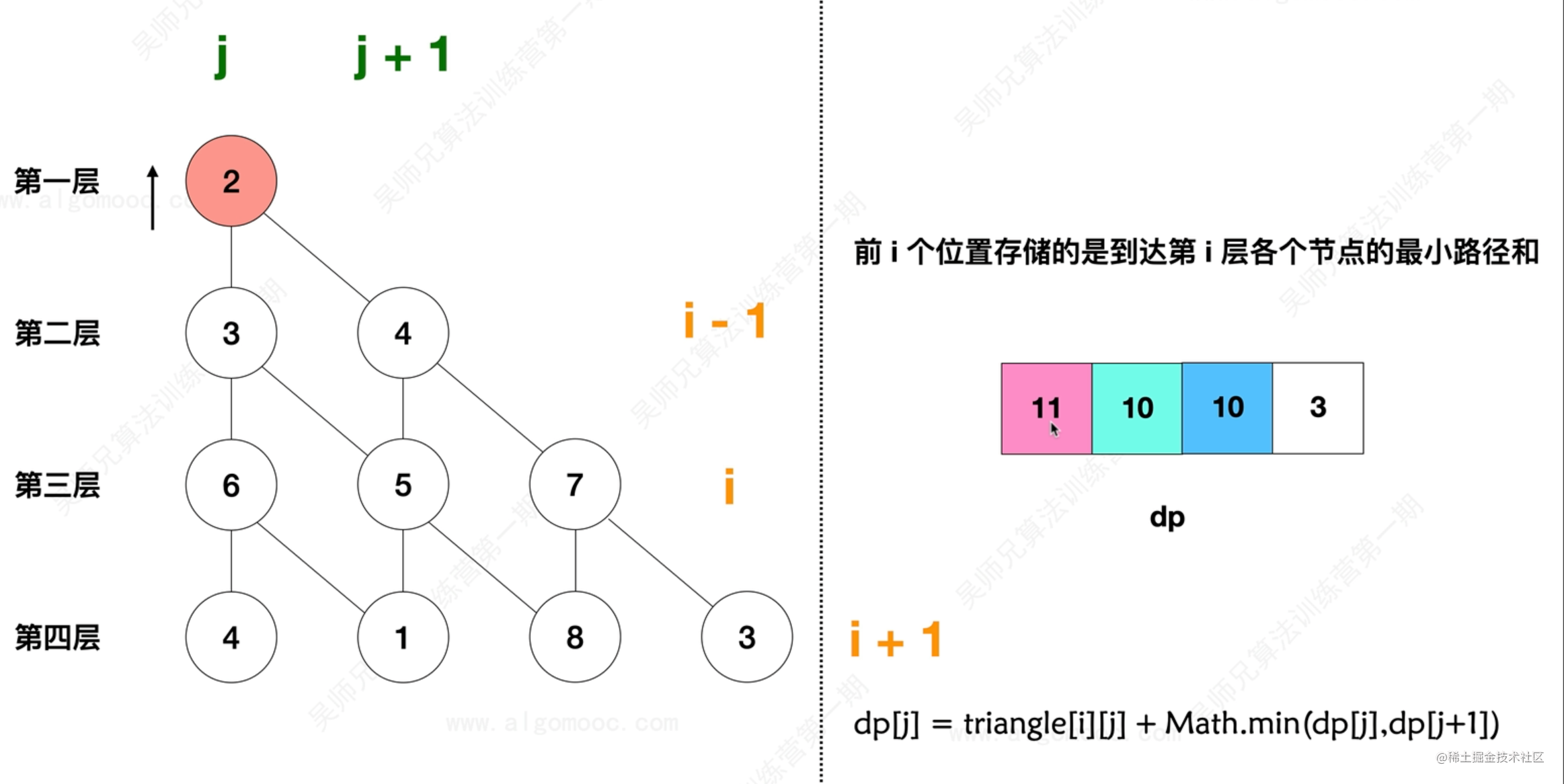``` class Solution { public int miniumTotal(List> triangle) // triangle 是个二维数组 // 先获取 triangle 的层数, 即一维数组的个数 int n = triangle.size();

``````    // 设置一个一维数组, 动态的更新每一层中当前结点对应的最短路径
int[] dp = new int[n + 1];

// 从最后一层开始计算结点的最短路径, 直到顶层 0层 为止
for (int i = n - 1; i >= 0; i--) {
// dp 中存储的是前 i 个位置存储的是到达第 i 层各个结点的最小路径和
// 从每一层的第 0 个位置开始
for (int j = 0; j <=i ; j++) {
// dp[j] 表示第 i 层中第 j 个结点的最小路径和
dp[j] = triangle.get(i).get(j) + Math.min(dp[j], dp[j+1]);
}
}

// 返回结果
return dp;
}
``````

} ``` - 力扣链接

#### 发文不易, 喜欢点赞的人更有好运气👍 :), 定期更新+关注不迷路~

ps：欢迎加入笔者18年建立的研究iOS审核及前沿技术的三千人扣群：662339934，坑位有限，备注“掘金网友”可被群管通过~